SNOS032I August   1999  – June 2016

PRODUCTION DATA.

1. Features
2. Applications
3. Description
4. Revision History
5. Pin Configuration and Functions
6. Specifications
7. Detailed Description
8. Application and Implementation
1. 8.1 Application Information
2. 8.2 Typical Applications
1. 8.2.1 Telephone-Line Transceiver
2. 8.2.2 “Simple” Mixer (Amplitude Modulator)
3. 8.2.3 Tri-Level Voltage Detector
4. 8.2.4 Dual Amplifier Active Filters (DAAFs)
3. 8.3 Do's and Don'ts
9. Power Supply Recommendations
10. 10Layout
11. 11Device and Documentation Support
12. 12Mechanical, Packaging, and Orderable Information

• DCK|5
• DBV|5
• DCK|5

6 Specifications

6.1 Absolute Maximum Ratings(1)(2)

MIN MAX UNIT
Differential Input Voltage V V+ V
Supply Voltage (V+– V) –0.3 5.5 V
Output Short Circuit to V+(3) See (3)
Output Short Circuit to V(3) See (3)
Soldering Information
Infrared or Convection (20 sec) 235 °C
Junction Temperature(1) 150 °C
Storage Temperature Tstg –65 150 °C
Absolute Maximum Ratings indicate limits beyond which damage to the device may occur. Operating Ratings indicate conditions for which the device is intended to be functional, but specific performance is not ensured. For ensured specifications and the test conditions, see the Electrical Characteristics.
Applies to both single-supply and split-supply operation. Continuous short circuit operation at elevated ambient temperature can result in exceeding the maximum allowed junction temperature of 150°C. Output currents in excess of 45 mA over long term may adversely affect reliability.

6.2 ESD Ratings

VALUE UNIT
V(ESD) Electrostatic discharge Human body model (HBM), per ANSI/ESDA/JEDEC JS-001, all pins(1) (2)(3) ±2000 V
Human body model (HBM), per ANSI/ESDA/JEDEC JS-001, all pins (1) LMV821 ±1500
Machine Model (MM) (4) ±200
Level listed above is the passing level per ANSI, ESDA, and JEDEC JS-001. JEDEC document JEP155 states that 500-V HBM allows safe manufacturing with a standard ESD control process.
Human body model, 1.5 kΩ in series wth 100 pF.
AEC Q100-002 indicates HBM stressing is done in accordance with the ANSI/ESDA/JEDEC JS-001 specification for Q grade devices.
Machine model, 200Ω in series with 100 pF.

6.3 Recommended Operating Conditions

MIN NOM MAX UNIT
Supply Voltage 2.5 5.5 V
Temperature Range LMV821, LMV822, LMV824 –40 85 °C
LMV822-Q1, LMV824I and LMV824-Q1 –40 125

6.4 Thermal Information, 5 Pins(1)

THERMAL METRIC(1) DCK
SC70-5 PACKAGE
DBV
SOT23-5 PACKAGE
UNIT
5 PIN 5 PIN
RθJA Junction-to-ambient thermal resistance 263.4 217.8 °C/W
RθJC(top) Junction-to-case (top) thermal resistance 102.8 142.4 °C/W
RθJB Junction-to-board thermal resistance 50.9 49.4 °C/W
ψJT Junction-to-top characterization parameter 3.7 29.1 °C/W
ψJB Junction-to-board characterization parameter 50.2 48.5 °C/W
RθJC(bot) Junction-to-case (bottom) thermal resistance N/A N/A °C/W

6.5 Thermal Information, 8 Pins(1)

THERMAL METRIC(1) D
SOIC PACKAGE
DGK
VSSOP PACKAGE
UNIT
8 PIN 8 PIN
RθJA Junction-to-ambient thermal resistance 132.6 193.9 °C/W
RθJC(top) Junction-to-case (top) thermal resistance 76.9 84.4 °C/W
RθJB Junction-to-board thermal resistance 73.2 114.5 °C/W
ψJT Junction-to-top characterization parameter 25.0 21.6 °C/W
ψJB Junction-to-board characterization parameter 72.6 113.0 °C/W
RθJC(bot) Junction-to-case (bottom) thermal resistance N/A N/A °C/W

6.6 Thermal Information, 14 Pins(1)

THERMAL METRIC(1) D
SOIC PACKAGE
PW
TSSOP PACKAGE
DGV
TVSOP PACKAGE
UNIT
14 PIN 14 PIN 14 PIN
RθJA Junction-to-ambient thermal resistance 109.7 135.6 148.2 °C/W
RθJC(top) Junction-to-case (top) thermal resistance 65.9 63.8 67.3 °C/W
RθJB Junction-to-board thermal resistance 64.1 77.4 77.5 °C/W
ψJT Junction-to-top characterization parameter 24.5 13.0 12.9 °C/W
ψJB Junction-to-board characterization parameter 63.9 76.8 76.9 °C/W
RθJC(bot) Junction-to-case (bottom) thermal resistance N/A N/A N/A °C/W

6.7 DC Electrical Characteristics 2.7V

Unless otherwise specified, all limits ensured for TJ = 25°C. V+ = 2.7V, V = 0V, VCM = 1.0V, VO = 1.35V and RL > 1 MΩ. Temperature extremes are −40°C ≤ TJ ≤ 85°C for LMV821/822/824, and −40°C ≤ TJ ≤ 125°C for LMV822-Q1/LMV824-Q1/LMV824I.
PARAMETER TEST CONDITIONS MIN (3) TYP (2) MAX (3) UNIT
VOS Input Offset Voltage LMV821/822/822-Q1/824 1 3.5 mV
LMV821/822/822-Q1/824, Over Temperature 4
LMV824-Q1/LMV824I 1
LMV824-Q1/LMV824I, Over Tempeature 5.5
TCVOS Input Offset Voltage Average Drift 1 μV/°C
IB Input Bias Current 30 90 nA
Over Temperature 140
IOS Input Offset Current 0.5 30 nA
Over Temperature 50
CMRR Common Mode Rejection Ratio 0V ≤ VCM ≤ 1.7V 70 85 dB
0V ≤ VCM ≤ 1.7V, Over Temperature 68
+PSRR Positive Power Supply Rejection Ratio 1.7V ≤ V+ ≤ 4V, V- = 1V, VO = 0V, VCM = 0V
LMV821/822/824/824-Q1/LMV824I
75 85 dB
1.7V ≤ V+ ≤ 4V, V- = 1V, VO = 0V, VCM = 0V
LMV821/822/824/824-Q1/LMV824I, Over Temperature
70
LMV822-Q1 75 85
−PSRR Negative Power Supply Rejection Ratio -1.0V ≤ V- ≤ -3.3V, V+ = 1.7V, VO = 0V, VCM = 0V
LMV821/822/824/824-Q1/LMV824I
73 85 dB
-1.0V ≤ V- ≤ -3.3V, V+ = 1.7V, VO = 0V, VCM = 0V
LMV821/822/824/824-Q1/LMV824I, Over Temperature
70
LMV822-Q1 73 85
VCM Input Common-Mode Voltage Range For CMRR ≥ 50dB –0.3 –0.2 V
1.9 2.0
AV Large Signal Voltage Gain Sourcing, RL = 600Ω to 1.35V,
VO = 1.35V to 2.2V;
LMV821/822/824
90 100 dB
Sourcing, RL = 600Ω to 1.35V,
VO = 1.35V to 2.2V;
LMV821/822/824, Over Temperature
85
LMV822-Q1/LMV824-Q1/LMV824I 90 100
Sinking, RL = 600Ω to 1.35V,
VO = 1.35V to 0.5V
LMV821/822/824
85 90 dB
Sinking, RL = 600Ω to 1.35V,
VO = 1.35V to 0.5V
LMV821/822/824, Over Temperature
80
LMV824I 85 90
LMV824I, Over Temperature 78
LMV822-Q1/LMV824-Q1 85 90
Sourcing, RL =2kΩ to 1.35V,
VO = 1.35V to 2.2V;
LMV821/822/824
95 100 dB
Sourcing, RL =2kΩ to 1.35V,
VO = 1.35V to 2.2V;
LMV821/822/824, Over Temperature
90
LMV822-Q1/LMV824-Q1/LMV824I 95 100
Sinking, RL = 2kΩ to 1.35V,
VO = 1.35V to 0.5V
LMV821/822/824
90 95 dB
Sinking, RL = 2kΩ to 1.35V,
VO = 1.35V to 0.5V
LMV821/822/824, Over Temperature
85
LMV822-Q1/LMV824-Q1/LMV824I 90 95
V O Output Swing V+ = 2.7V, RL= 600Ω to 1.35V 2.50 2.58 V
0.13 0.20
V+ = 2.7V, RL= 600Ω to 1.35V, Over Temp 2.40 0.30
V+ = 2.7V, RL= 2kΩ to 1.35V 2.60 2.66 V
0.08 0.120
V+ = 2.7V, RL= 2kΩ to 1.35V, Over Temp 2.50 0.200
IO Output Current Sourcing, VO = 0V 12 16 mA
Sinking, VO = 2.7V 12 26
IS Supply Current LMV821 (Single) 0.22 0.3 mA
LMV821, Over Temperature 0.5
LMV822 (Dual) 0.45 0.6 mA
LMV822, Over Temperature 0.8
LMV824, Over Temperature 1.2

6.8 DC Electrical Characteristics 2.5V

Unless otherwise specified, all limits ensured for TJ = 25°C. V+ = 2.5V, V = 0V, VCM = 1.0V, VO = 1.25V and RL > 1 MΩ. Temperature extremes are −40°C ≤ TJ ≤ 85°C for LMV821/822/824, and −40°C ≤ TJ ≤ 125°C for LMV822-Q1/LMV824-Q1/LMV824I.
PARAMETER CONDITION MIN (3) TYP (2) MAX (3) UNIT
VOS Input Offset Voltage LMV821/822/822-Q1/824 1 3.5 mV
LMV821/822/822-Q1/824, Over Temperature 4
LMV824-Q1/LMV824I 1
LMV824-Q1/LMV824I, Over Temperature 5.5
V O Output Swing V+ = 2.5V, RL = 600Ω to 1.25V 2.30 2.37 V
0.13 0.20
V+ = 2.5V, RL = 600Ω to 1.25V, Over Temperature 2.20 0.30
V+ = 2.5V, RL = 2kΩ to 1.25V 2.40 2.46 V
0.08 0.12
V+ = 2.5V, RL = 2kΩ to 1.25V, Over Temperature 2.30 0.20

6.9 AC Electrical Characteristics 2.7V

Unless otherwise specified, all limits ensured for TJ = 25°C. V+ = 2.7V, V = 0V, VCM = 1.0V, VO = 1.35V and RL > 1 MΩ. Temperature extremes are −40°C ≤ TJ ≤ 85°C for LMV821/822/824, and −40°C ≤ TJ ≤ 125°C for LMV822-Q1/LMV824-Q1/LMV824I.
PARAMETER TEST CONDITIONS MIN (3) TYP (2) MAX (3) UNIT
SR Slew Rate See (4) 1.5 V/μs
GBW Gain-Bandwdth Product 5 MHz
Φm Phase Margin 61 Deg.
Gm Gain Margin 10 dB
Amp-to-Amp Isolation See (5) 135 dB
en Input-Related Voltage Noise f = 1 kHz, VCM = 1V 28 nV/√Hz
in Input-Referred Current Noise f = 1 kHz 0.1 pA/√Hz
THD Total Harmonic Distortion f = 1 kHz, AV = −2,
RL = 10 kΩ, VO = 4.1 V PP
0.01%

6.10 DC Electrical Characteristics 5V

Unless otherwise specified, all limits ensured for TJ = 25°C. V+ = 5 V, V = 0V, VCM = 2.0V, VO = 2.5V and RL > 1 MΩ. Temperature extremes are −40°C ≤ TJ ≤ 85°C for LMV821/822/824, and −40°C ≤ TJ ≤ 125°C for LMV822-Q1/LMV824-Q1/LMV824I.
PARAMETER TEST CONDITIONS MIN (3) TYP (2) MAX (3) UNIT
VOS Input Offset Voltage LMV821/822/822-Q1/824 1 3.5 mV
LMV821/822/822-Q1/824, Over Temperature 4.0
LMV824-Q1/LMV824I 1
LMV824-Q1/ LMV824I, Over Temperature 5.5
TCVOS Input Offset Voltage Average Drift 1 μV/°C
IB Input Bias Current 40 100 nA
Over Temperature 150
IOS Input Offset Current 0.5 30 nA
Over Temperature 50
CMRR Common Mode Rejection Ratio 0V ≤ VCM ≤ 4.0V 72 90 dB
0V ≤ VCM ≤ 4.0V, Over Temperature 70
+PSRR Positive Power Supply Rejection Ratio 1.7V ≤ V+ ≤ 4V, V- = 1V, VO = 0V, VCM = 0V
LMV821/822/824/824-Q1/824I
85 75 dB
1.7V ≤ V+ ≤ 4V, V- = 1V, VO = 0V, VCM = 0V
LMV821/822/824/824-Q1/824I, Over Temperature
70
LMV822-Q1 75 85
−PSRR Negative Power Supply Rejection Ratio -1.0V ≤ V- ≤ -3.3V, V+ = 1.7V, VO = 0V, VCM = 0V
LMV821/822/824/824-Q1/824I
73 85 dB
-1.0V ≤ V- ≤ -3.3V, V+ = 1.7V, VO = 0V, VCM = 0V
LMV821/822/824/824-Q1/824I
70
LMV822-Q1 73 85
VCM Input Common-Mode Voltage Range For CMRR ≥ 50dB -0.3 -0.2 V
4.2 4.3 V
AV Large Signal Voltage Gain Sourcing, RL = 600Ω to 2.5V,
VO = 2.5V to 4.5V;
LMV821/822/824
95 105 dB
Sourcing, RL = 600Ω to 2.5V,
VO = 2.5V to 4.5V;
LMV821/822/824, Over Temperature
90
LMV822-Q1/LMV824-Q1/LMV824I 95 105
Sinking, RL = 600Ω to 2.5V,
VO = 2.5V to 0.5V
LMV821/822/824
95 105 dB
Sinking, RL = 600Ω to 2.5V,
VO = 2.5V to 0.5V
LMV821/822/824, Over Temperature
90
LMV824I 95 105
LMV824I, Over Temperature 82
LMV822-Q1/LMV824-Q1 95 105
Sourcing, RL =2kΩ to 2.5V,
VO = 2.5V to 4.5V;
LMV821/822/824
95 105 dB
Sourcing, RL =2kΩ to 2.5V,
VO = 2.5V to 4.5V;
LMV821/822/824, Over Temperature
90
LMV822-Q1/LMV824-Q1/LMV824I 95 105
Sinking, RL = 2kΩ to 2.5V,
VO = 2.5V to 0.5V
LMV821/822/824
95 105 dB
Sinking, RL = 2kΩ to 2.5V,
VO = 2.5V to 0.5V
LMV821/822/824, Over Temperature
90
LMV822-Q1/LMV824-Q1/LMV824I 95 105
V O Output Swing V+ = 5V,RL = 600Ω to 2.5V 4.75 4.84 V
V+ = 5V,RL = 600Ω to 2.5V, Over Temperature 4.70
V+ = 5V,RL = 600Ω to 2.5V (LMV824-Q1, LMV824I) 4.84
V+ = 5V,RL = 600Ω to 2.5V (LMV824-Q1, LMV824I), Over Temperature 4.60
V+ = 5V,RL = 600Ω to 2.5V 0.17 0.250 V
V+ = 5V,RL = 600Ω to 2.5V, Over Temperature 0.30
V+ = 5V,RL = 600Ω to 2.5V (LMV824-Q1, LMV824I) 0.17
V+ = 5V,RL = 600Ω to 2.5V (LMV824-Q1, LMV824I), Over Temperature 0.40
V+ = 5V, RL = 2kΩ to 2.5V 4.85 4.90 V
0.10 0.15
V+ = 5V, RL = 2kΩ to 2.5V, Over Temperature 4.80 0.20
IO Output Current Sourcing, VO = 0V 20 45 mA
Sourcing, VO = 0V, Over Temperature 15
Sourcing, VO = 0V
LMV824I
20 45 mA
Sourcing, VO = 0V
LMV824I, Over Temperature
10
Sinking, VO = 5V 20 40 mA
Sinking, VO = 5V, Over Temperature 15
Sinking, VO = 5V
LMV824I
20 40 mA
Sinking, VO = 5V
LMV824I, Over Temperature
10
IS Supply Current LMV821 (Single) 0.30 0.4 mA
LMV821, Over Temperature 0.6
LMV822 (Dual) 0.5 0.7 mA
LMV822, Over Temperature 0.9
LMV824, Over Temperature 1.5
LMV824I, Over Temperature 1.6

6.11 AC Electrical Characteristics 5V

Unless otherwise specified, all limits ensured for TJ = 25°C. V+ = 5 V, V = 0V, VCM = 2.0V, VO = 2.5V and RL > 1 MΩ. Temperature extremes are −40°C ≤ TJ ≤ 85°C for LMV821/822/824, and −40°C ≤ TJ ≤ 125°C for LMV822-Q1/LMV824-Q1/LMV824I.
PARAMETER TEST CONDITIONS MIN (3) TYP (2) MAX (3) UNIT
SR Slew Rate See (4) 1.4 2.0 V/μs min
GBW Gain-Bandwdth Product 5.6 MHz
Φm Phase Margin 67 Deg.
Gm Gain Margin 15 dB
Amp-to-Amp Isolation See (5) 135 dB
en Input-Related Voltage Noise f = 1 kHz, VCM = 1V 24 nV/√Hz
in Input-Referred Current Noise f = 1 kHz 0.25 pA/√Hz
THD Total Harmonic Distortion f = 1 kHz, AV = −2,
RL = 10 kΩ, VO = 4.1 V PP
0.01%
The maximum power dissipation is a function of TJ(max) , θJA, and TA. The maximum allowable power dissipation at any ambient temperature is PD = (TJ(max)–TA)/θJA. All numbers apply for packages soldered directly into a PC board.
Typical Values represent the most likely parametric norm.
All limits are ensured by testing or statistical analysis.
V+ = 5V. Connected as voltage follower with 3V step input. Number specified is the slower of the positive and negative slew rates.
Input referred, V+ = 5V and RL = 100kΩ connected to 2.5V. Each amp excited in turn with 1 kHz to produce VO = 3 VPP.

6.12 Typical Characteristics

Unless otherwise specified, VS = +5V, single supply, TA = 25°C.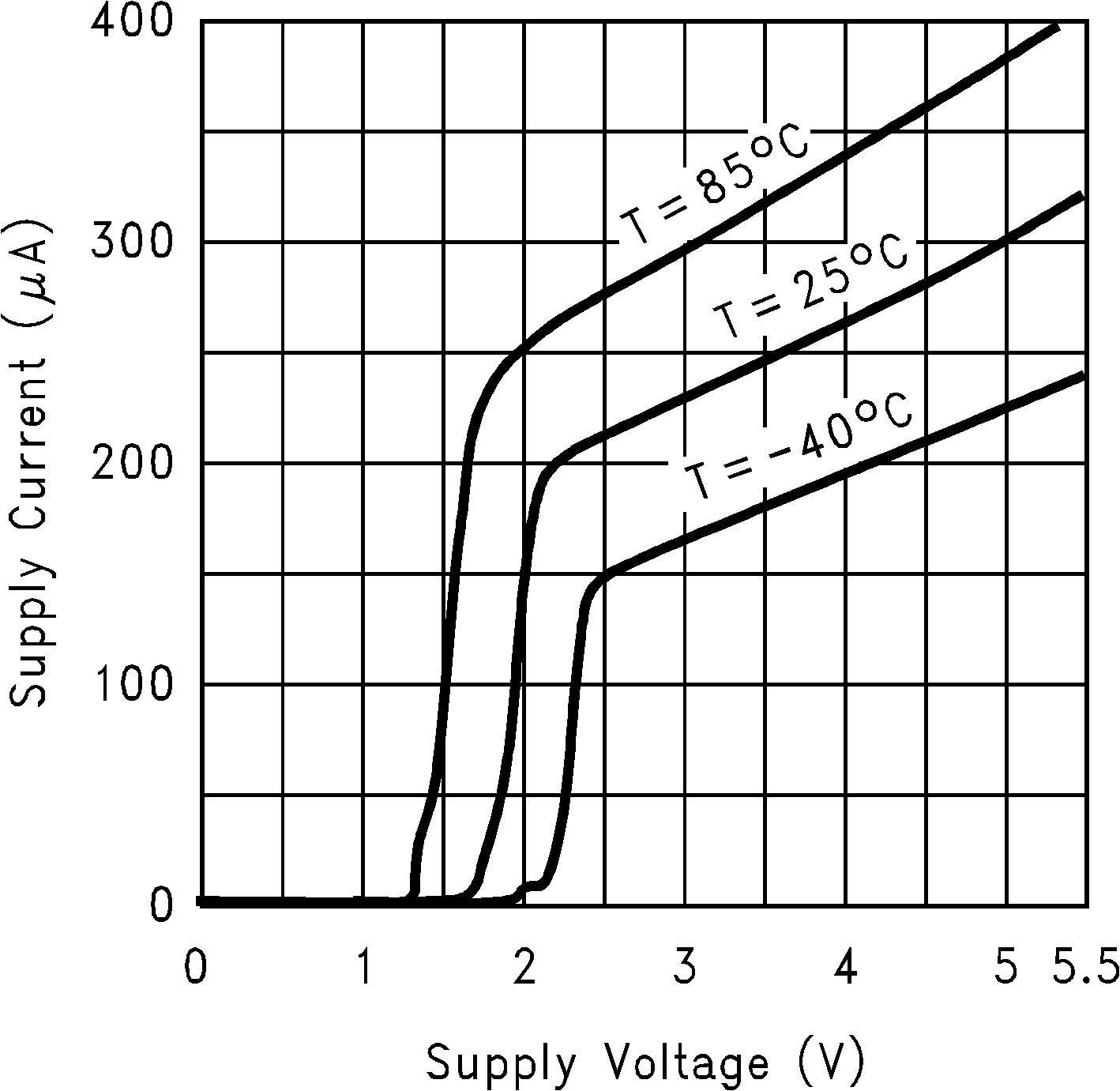Figure 1. Supply Current vs. Supply Voltage (LMV821)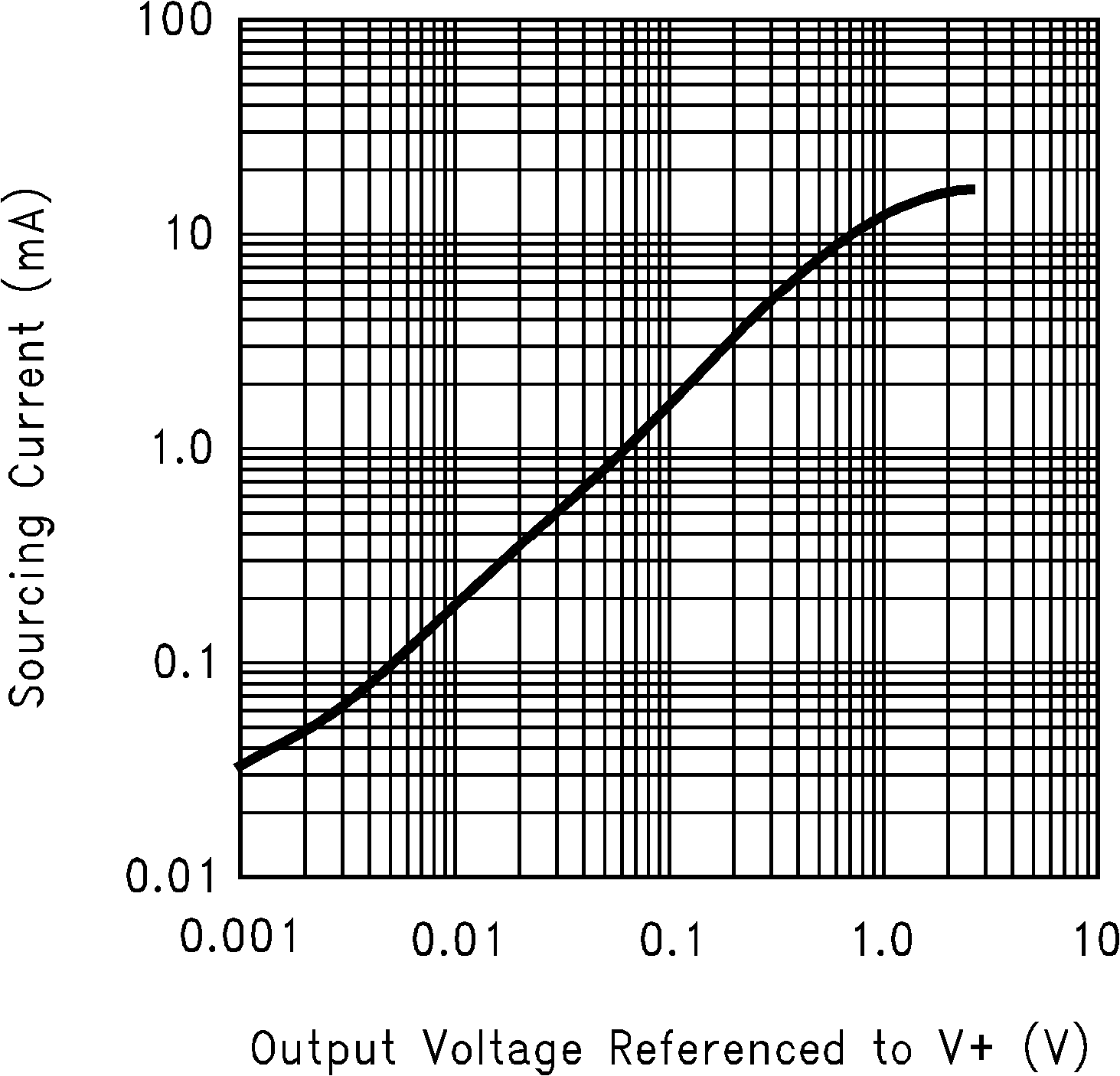Figure 3. Sourcing Current vs. Output Voltage (VS = 2.7V)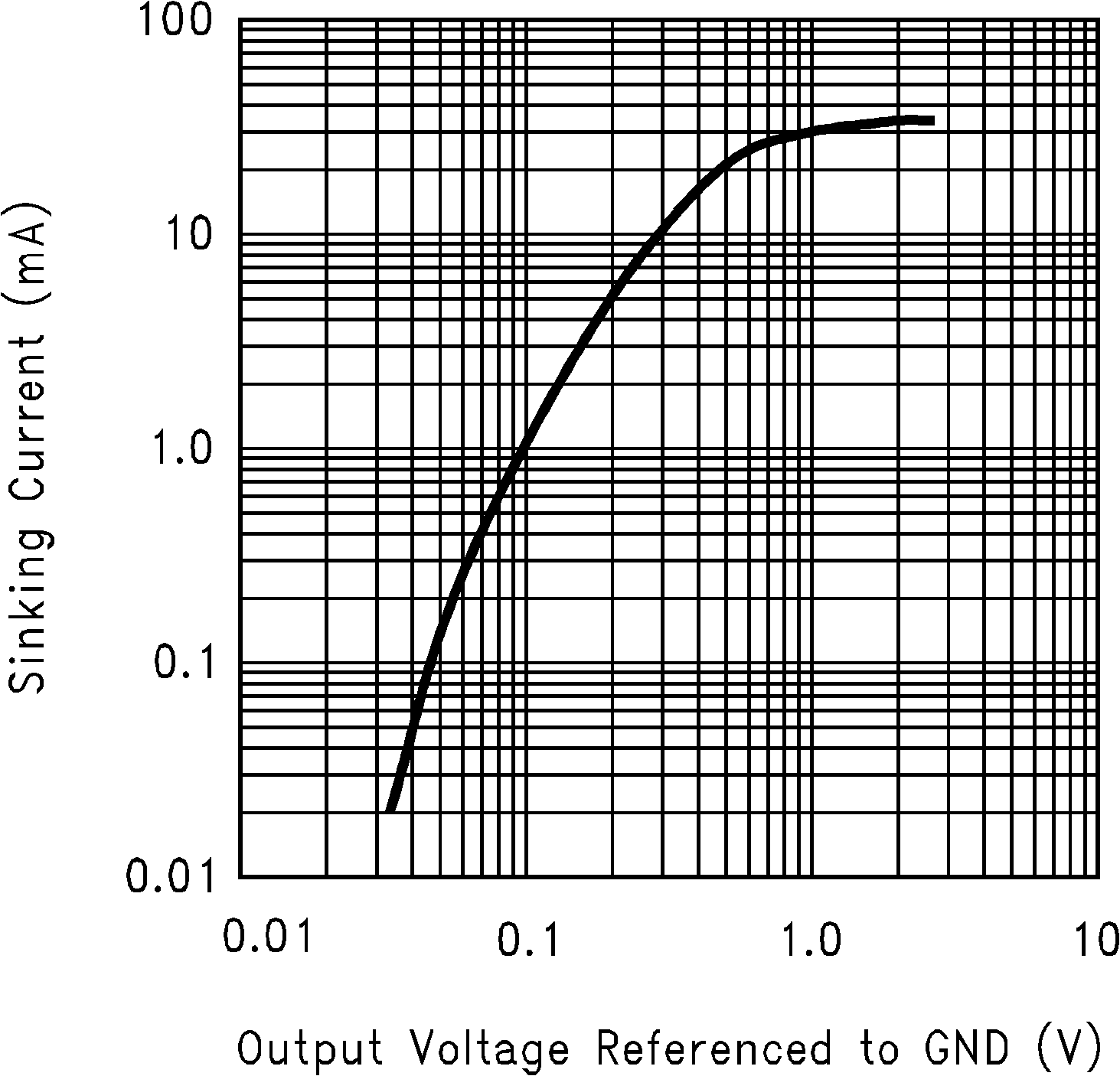Figure 5. Sinking Current vs. Output Voltage (VS = 2.7V)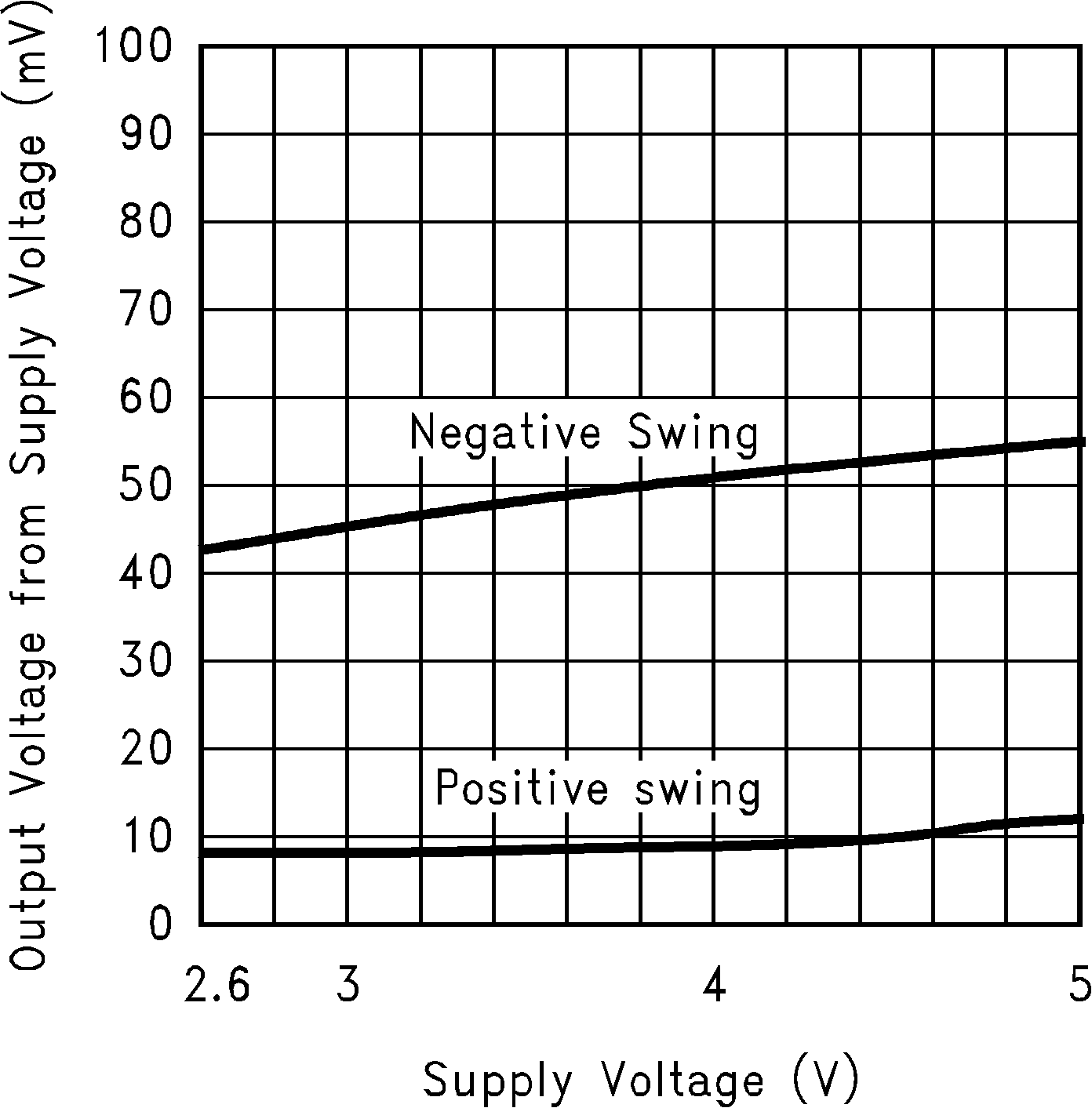Figure 7. Output Voltage Swing vs. Supply Voltage
(RL = 10kΩ)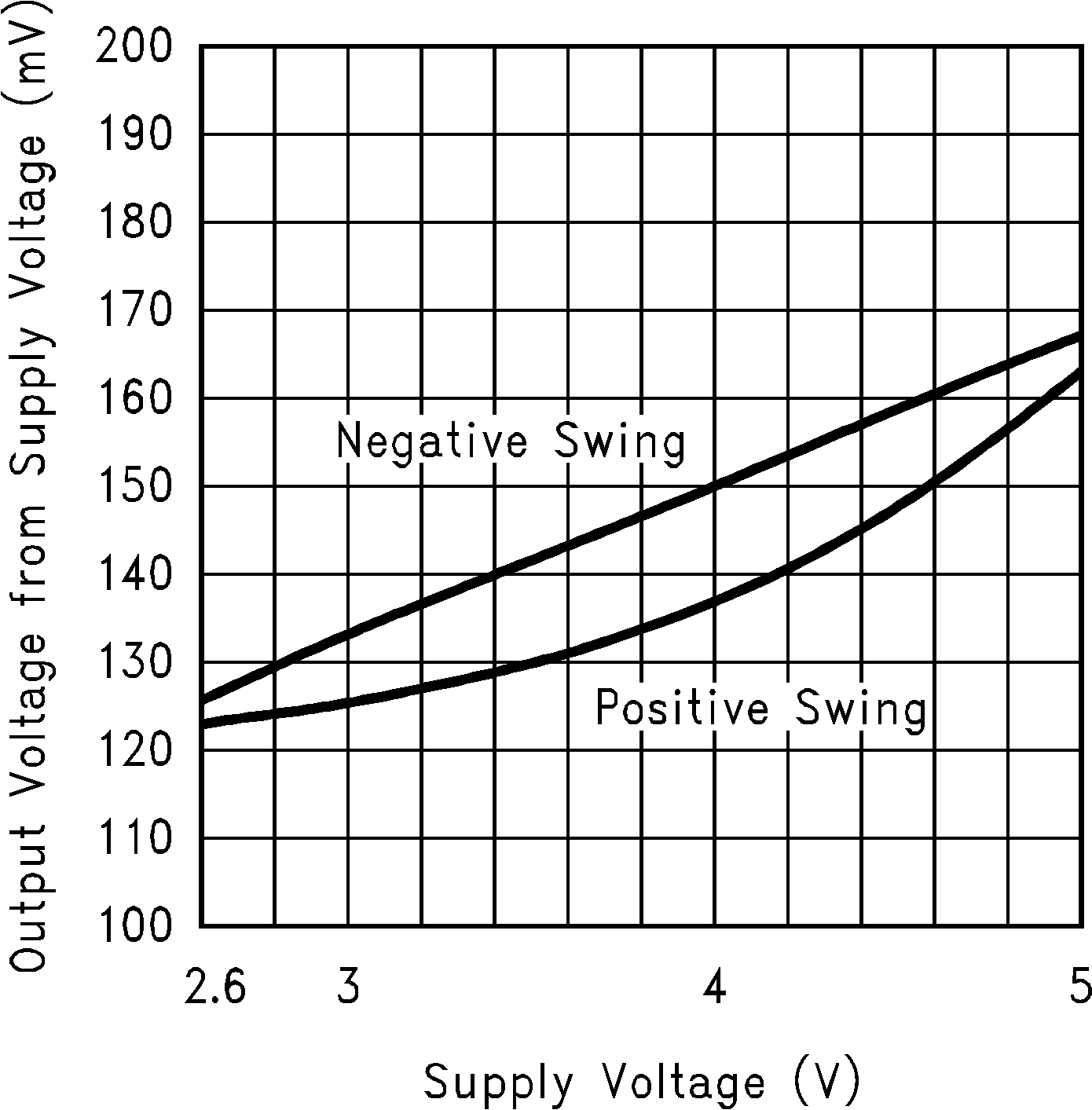Figure 9. Output Voltage Swing vs. Supply Voltage (RL = 600Ω)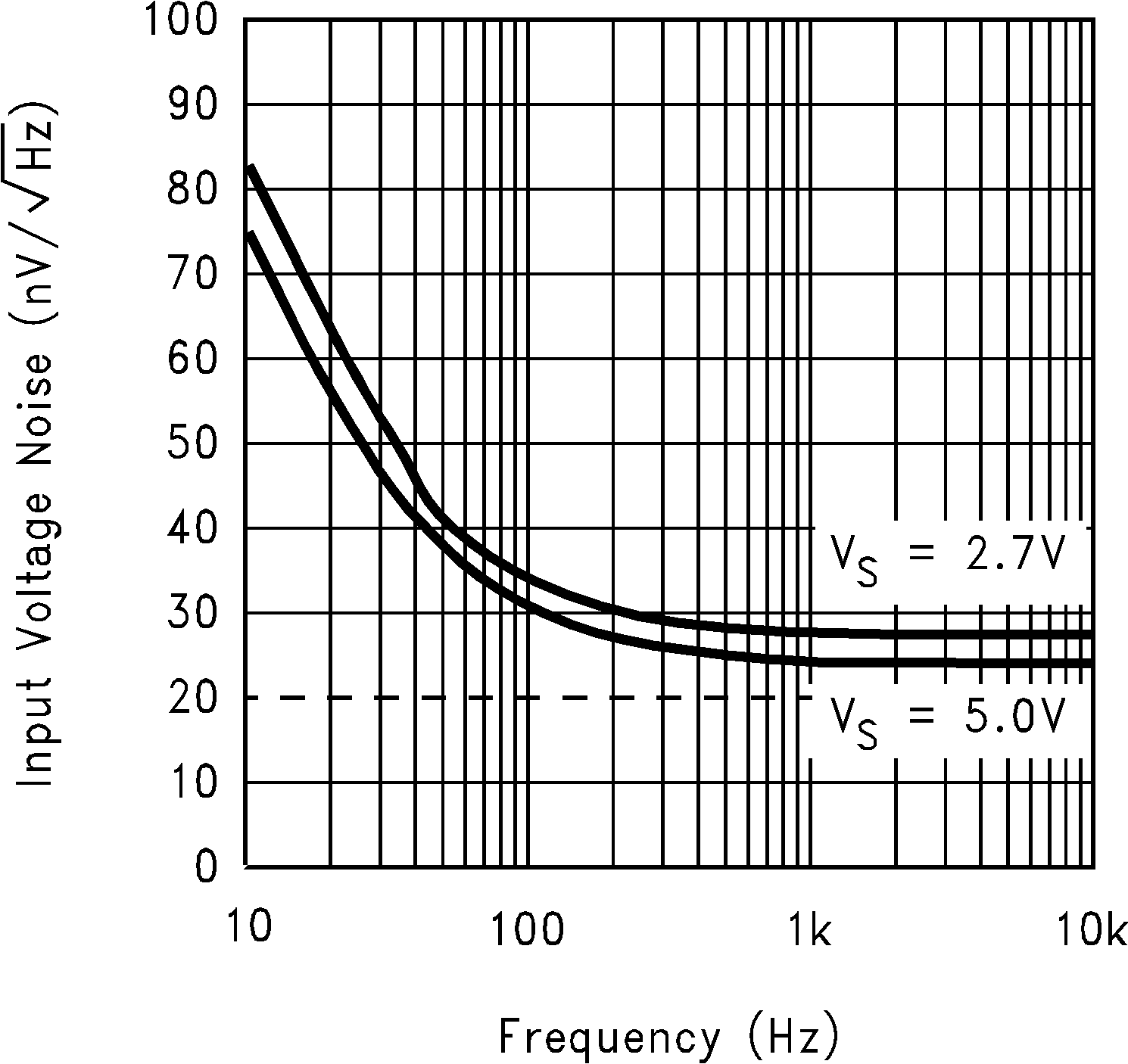Figure 11. Input Voltage Noise vs. Frequency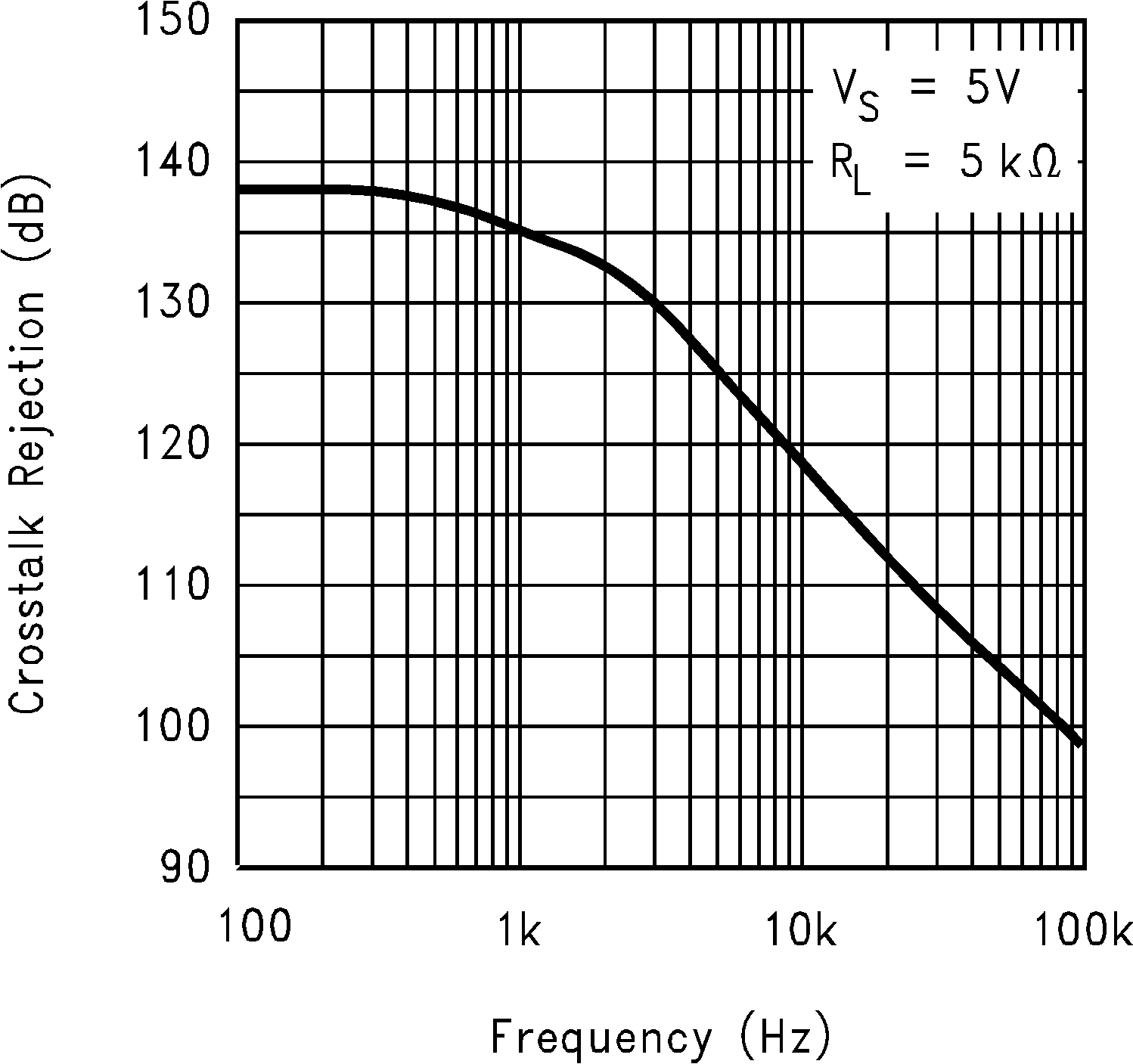Figure 13. Crosstalk Rejection vs. Frequency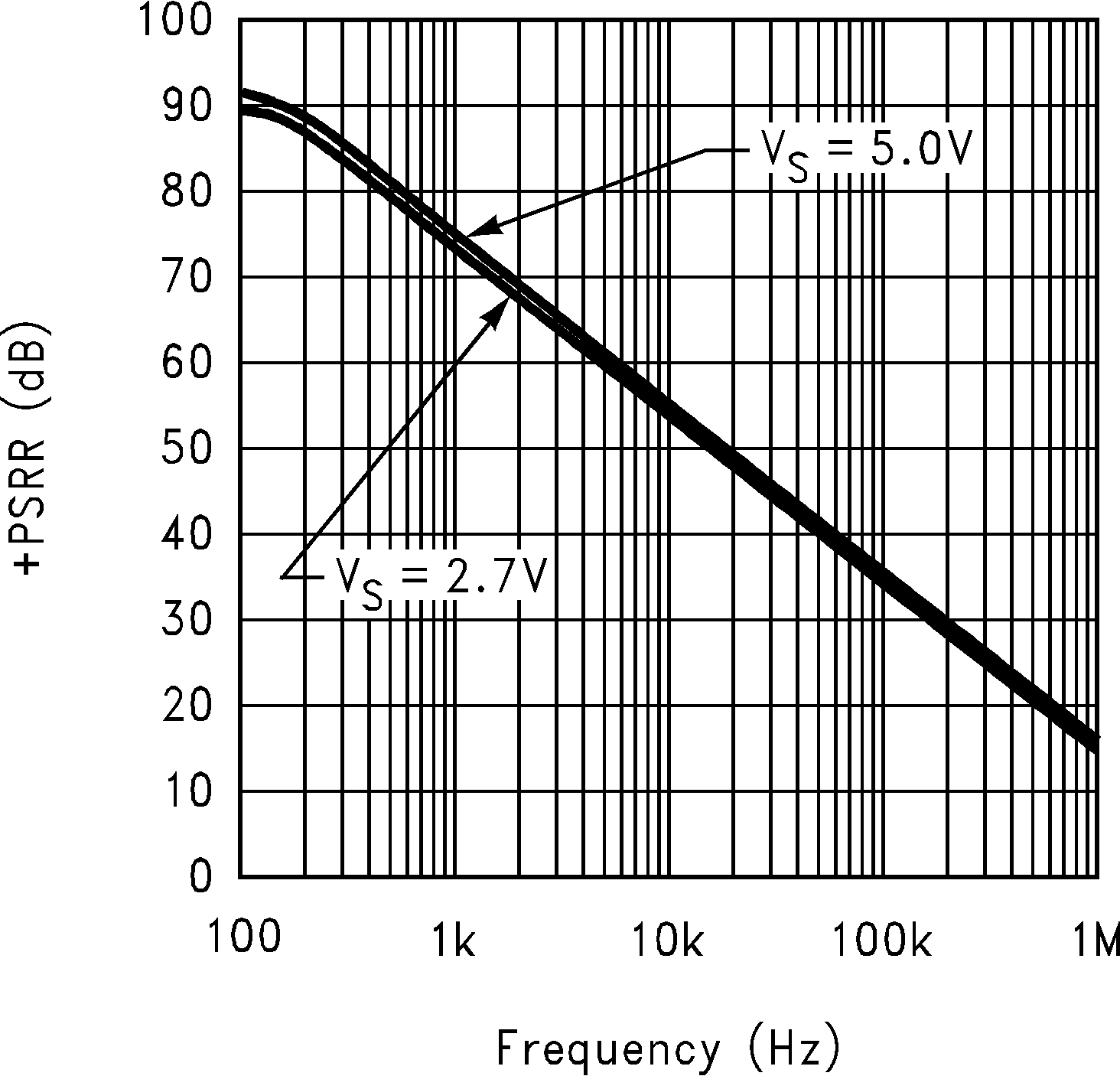Figure 15. -PSRR vs. Frequency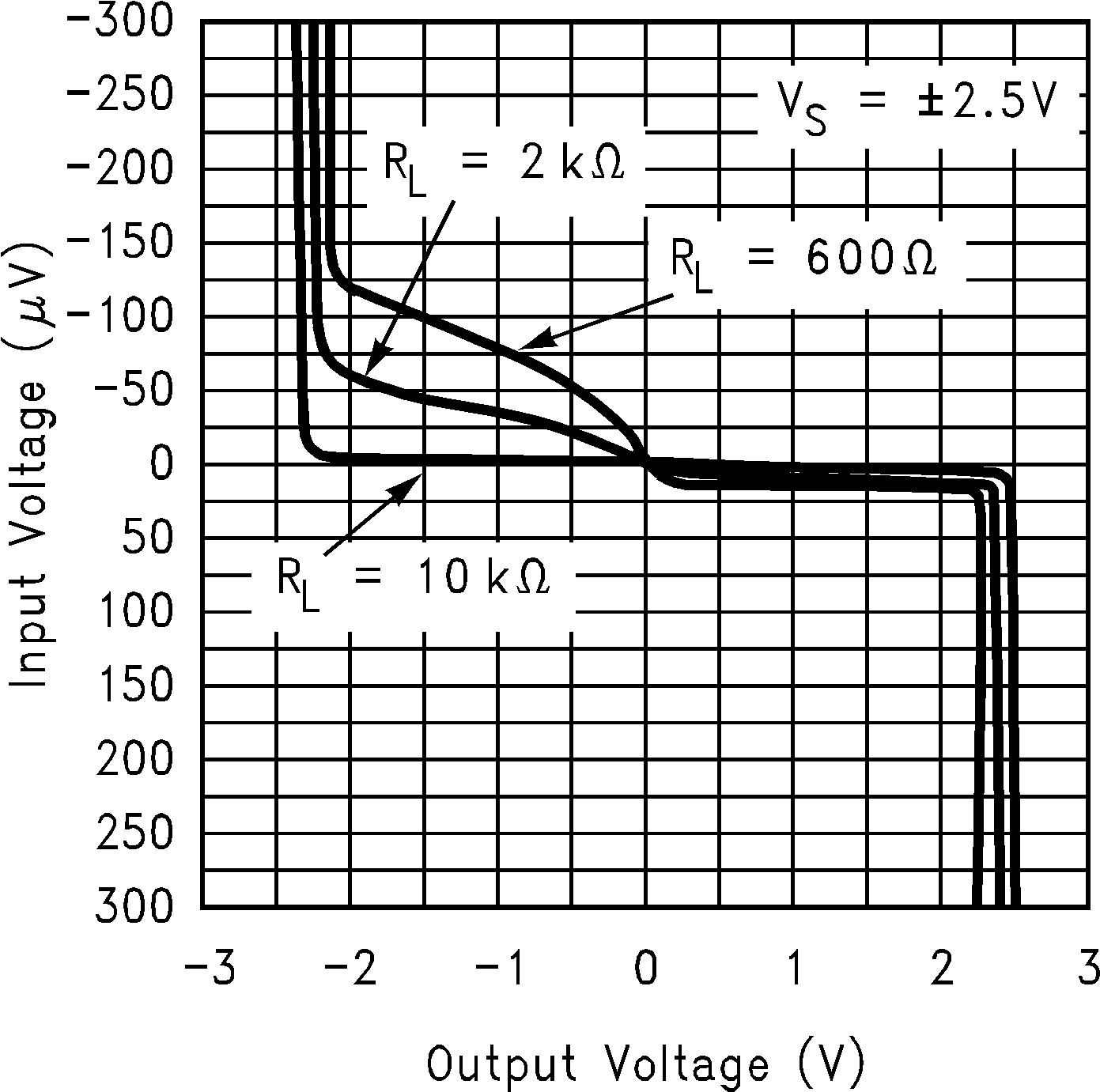Figure 17. Input Voltage vs. Output Voltage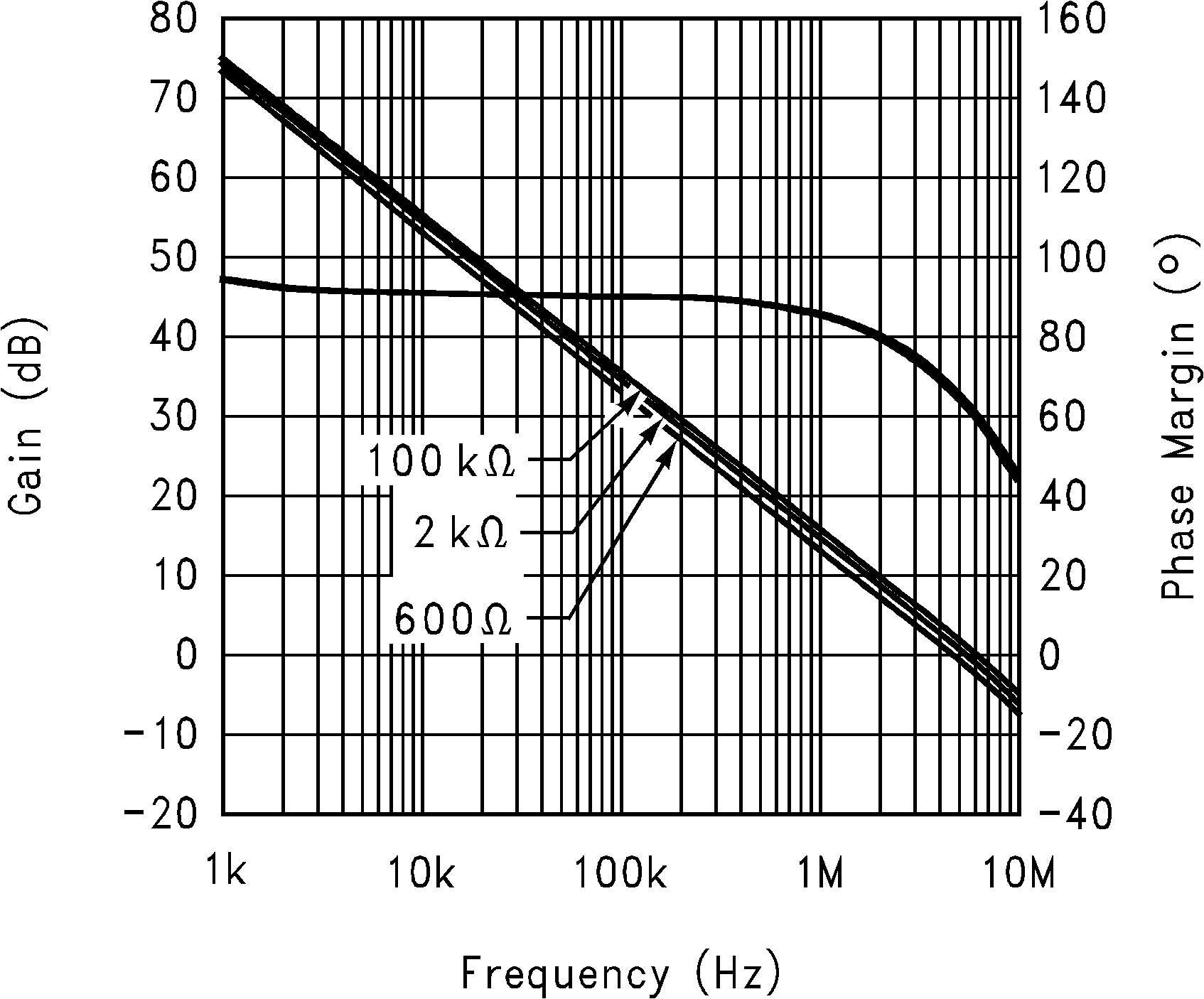Figure 19. Gain and Phase Margin vs. Frequency
(RL = 100kΩ, 2kΩ, 600Ω) at 5V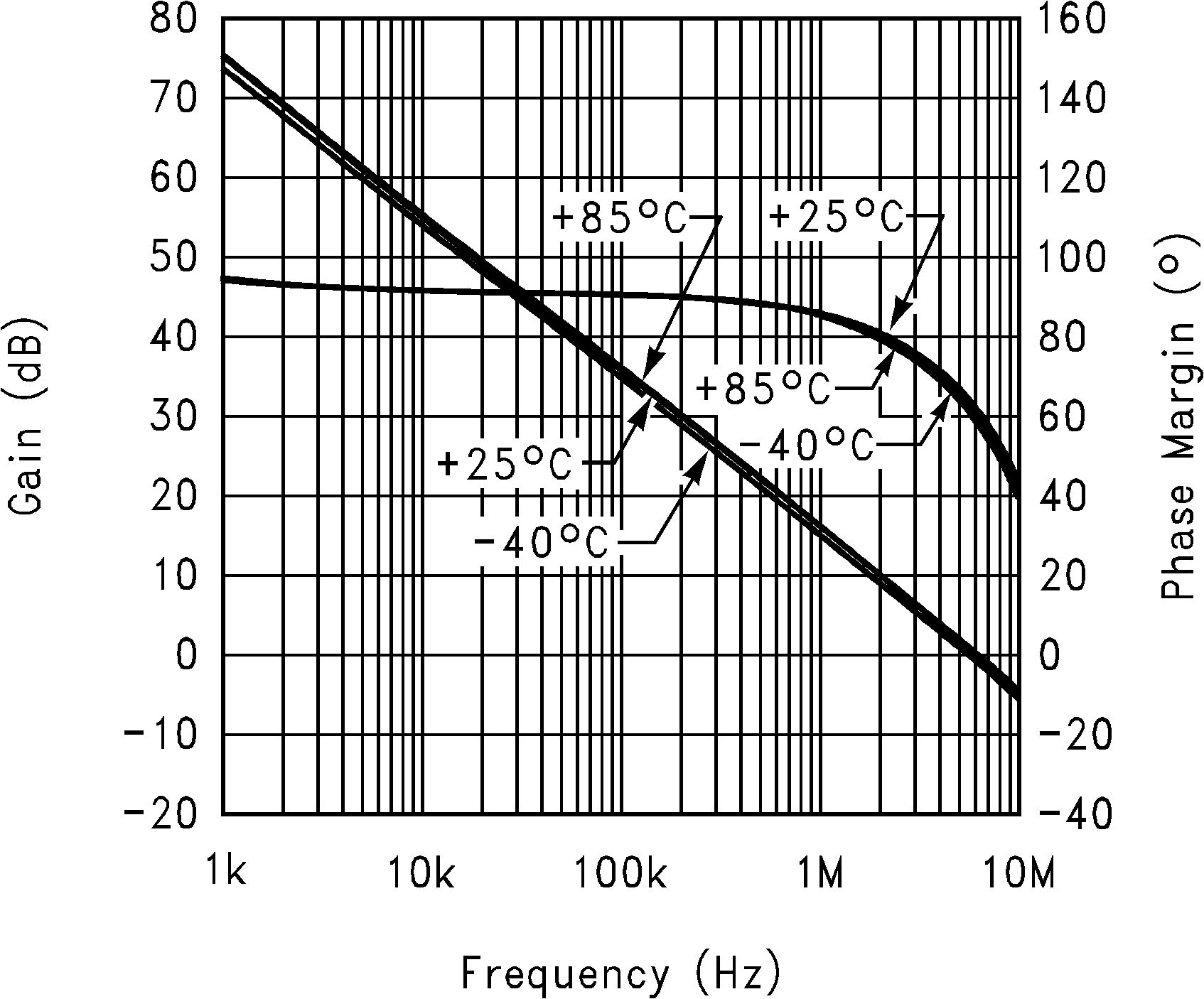Figure 21. Gain and Phase Margin vs. Frequency
(Temp.= 25, -40, 85 °C, RL = 10kΩ) at 5V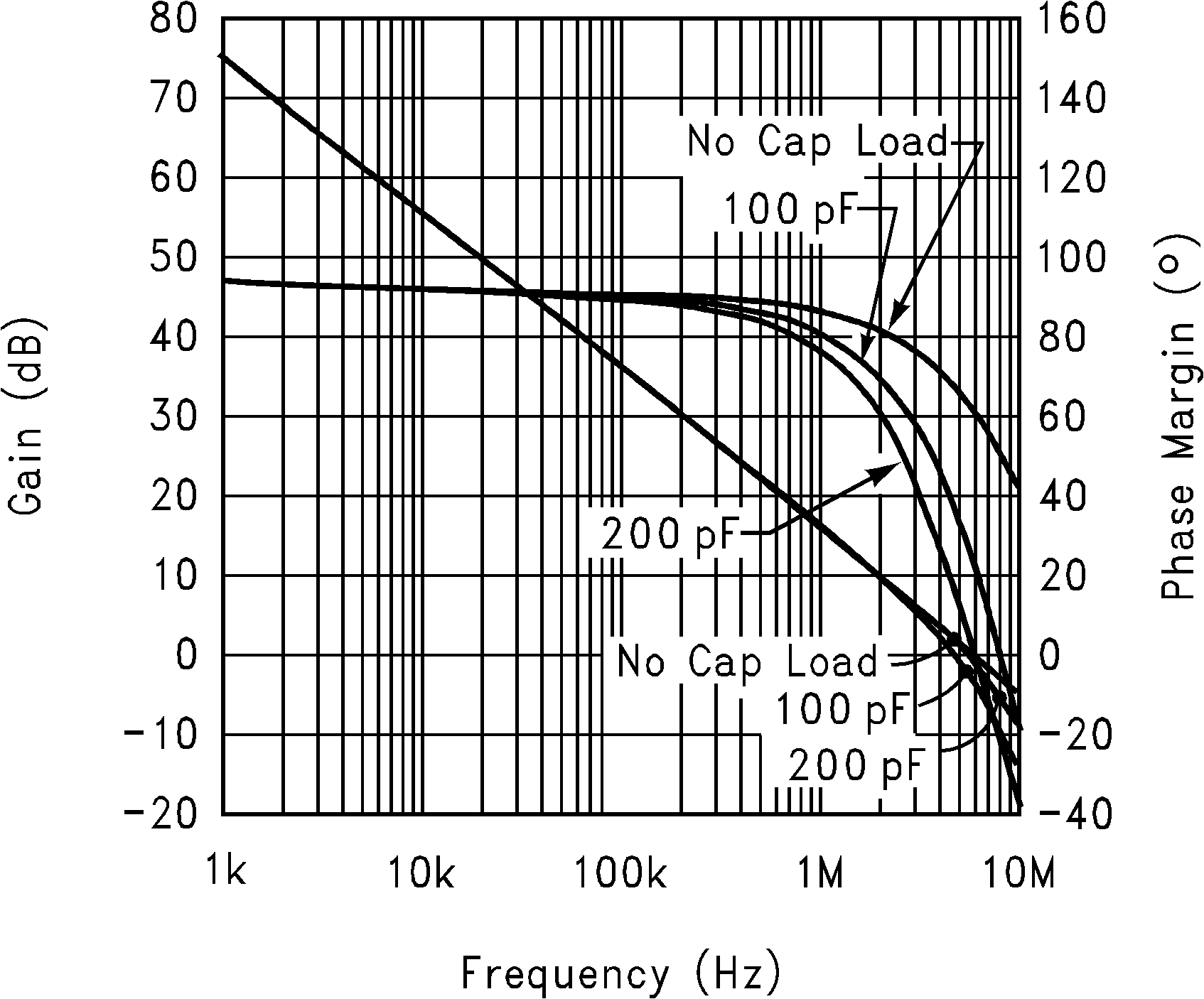Figure 23. Gain and Phase Margin vs. Frequency
(CL = 100pF, 200pF, 0pF RL = 10kΩ) at 5V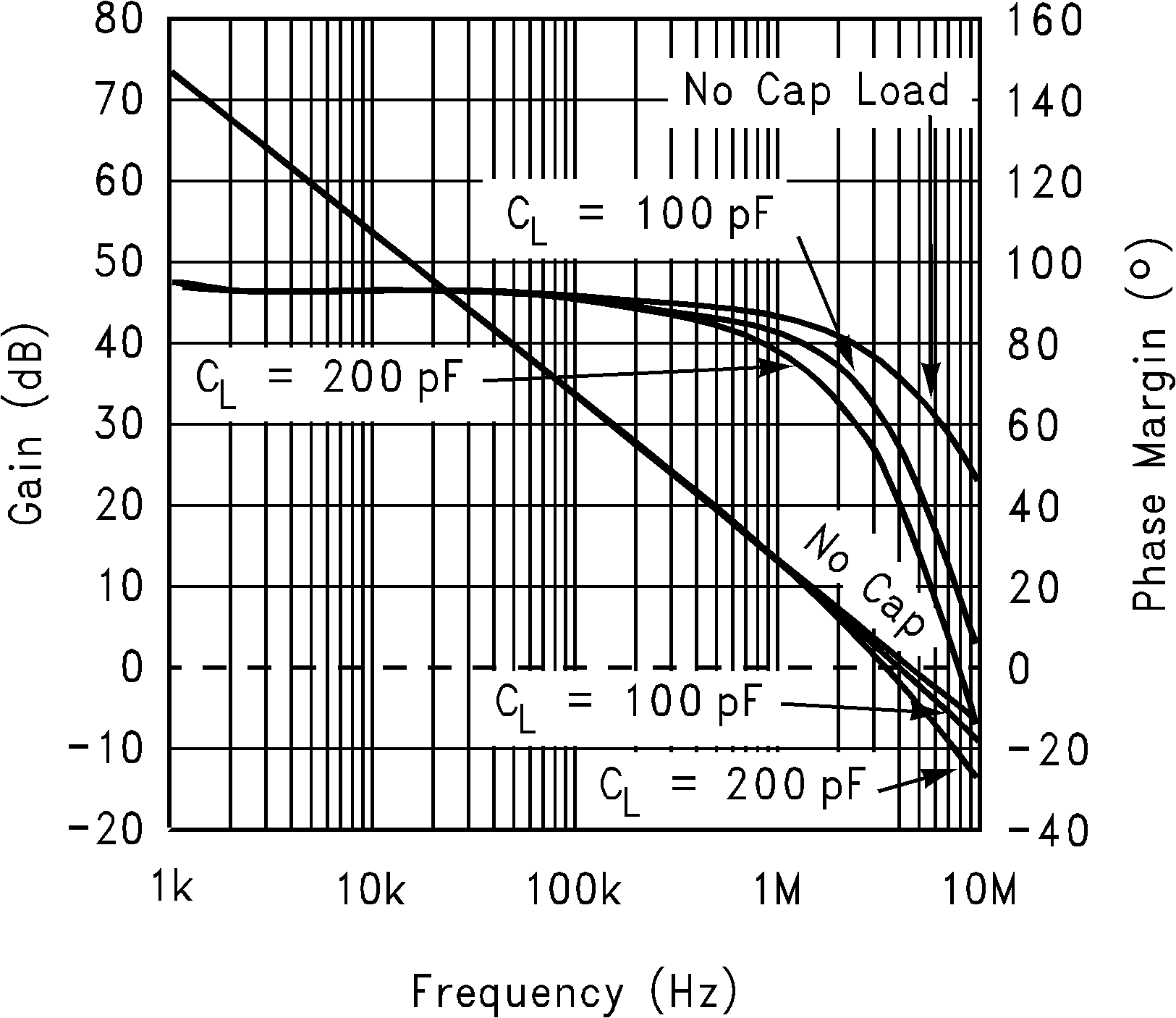Figure 25. Gain and Phase Margin vs. Frequency
(CL = 100pF, 200pF, 0pF RL = 600Ω) at 5V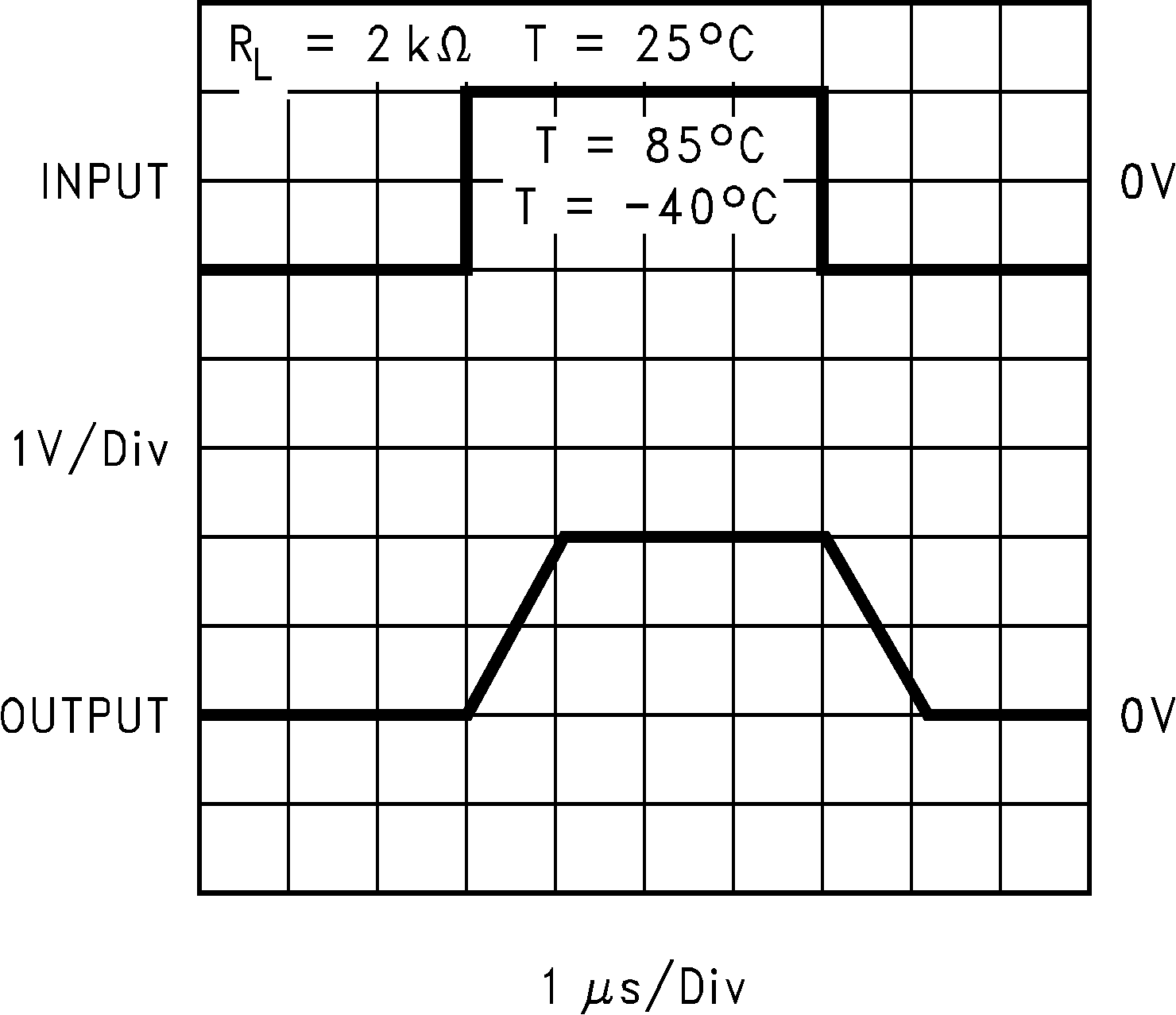Figure 27. Non-Inverting Large Signal Pulse ResponseFigure 29. Inverting Large Signal Pulse Response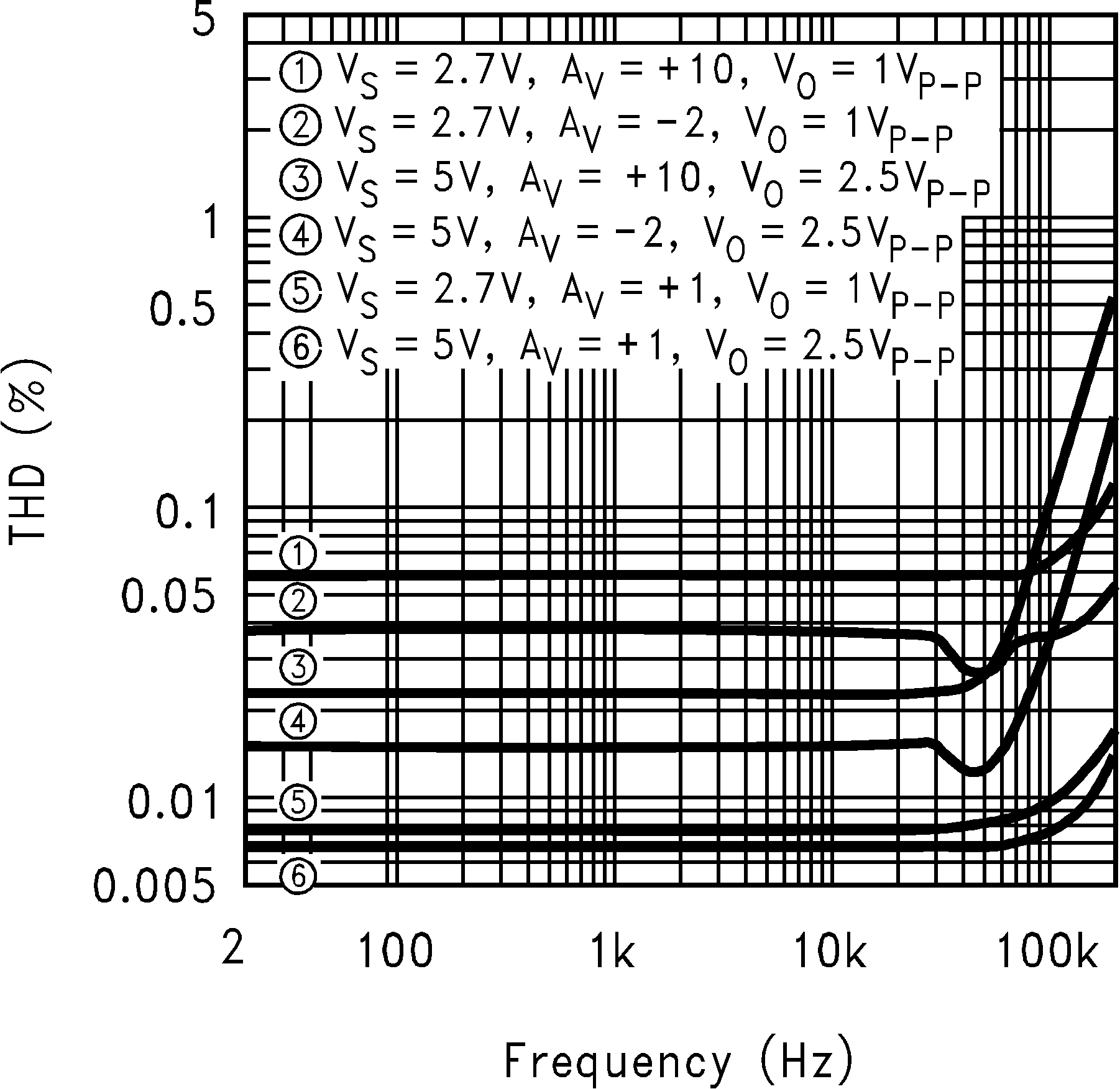Figure 31. THD vs. Frequency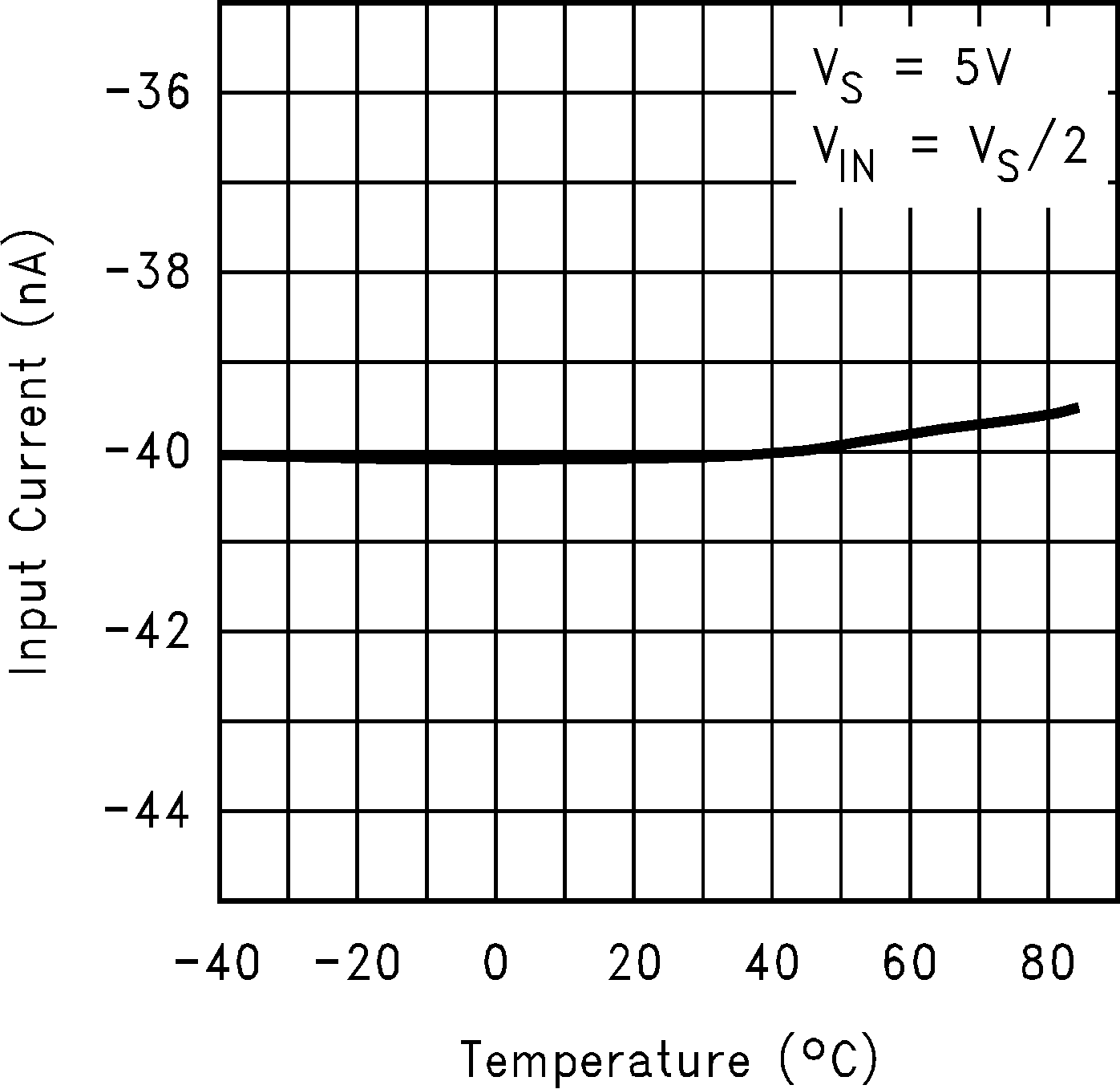Figure 2. Input Current vs. Temperature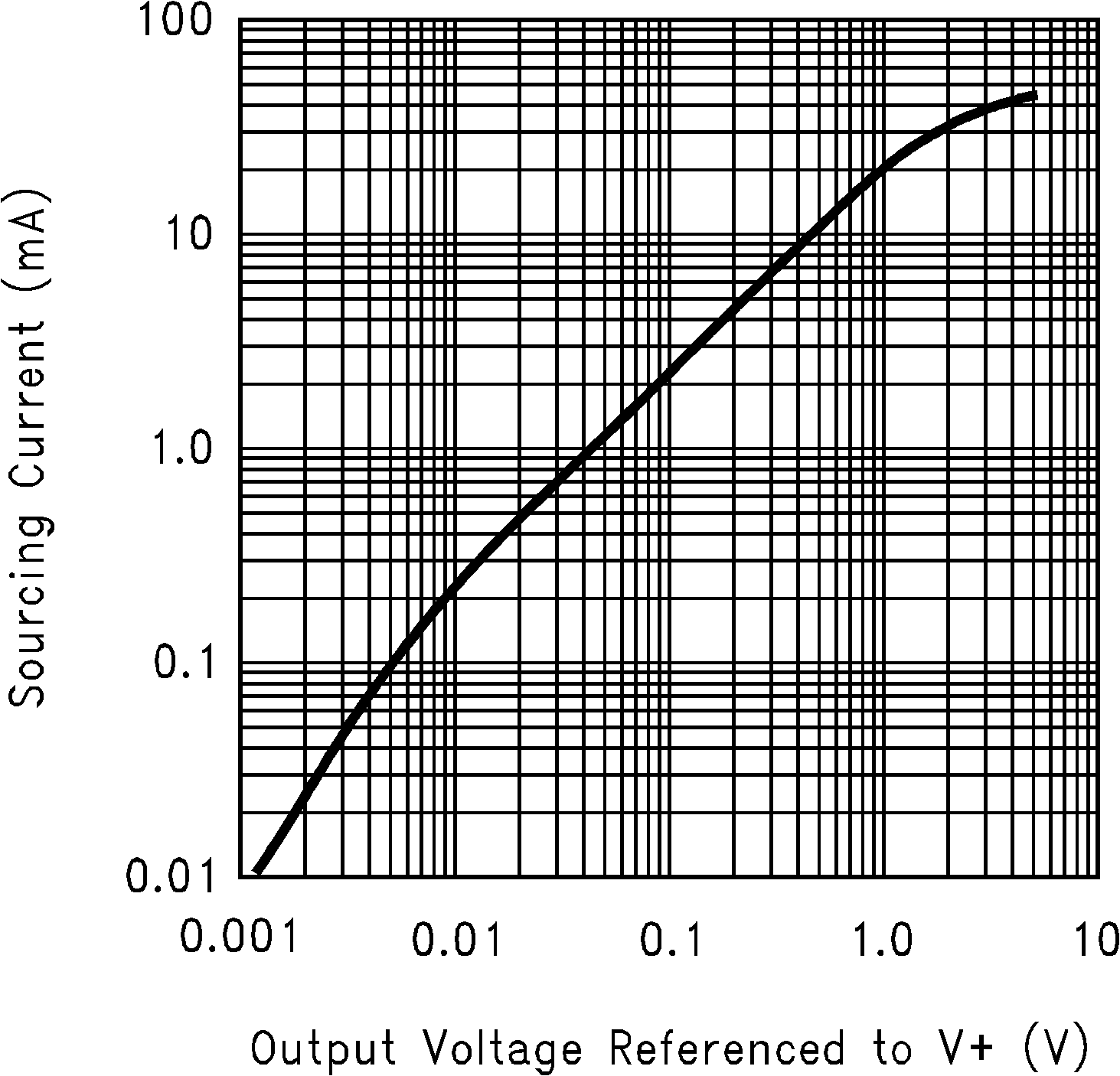Figure 4. Sourcing Current vs Output Voltage (VS = 5V)Figure 6. Sinking Current vs. Output Voltage
(VS = 5V)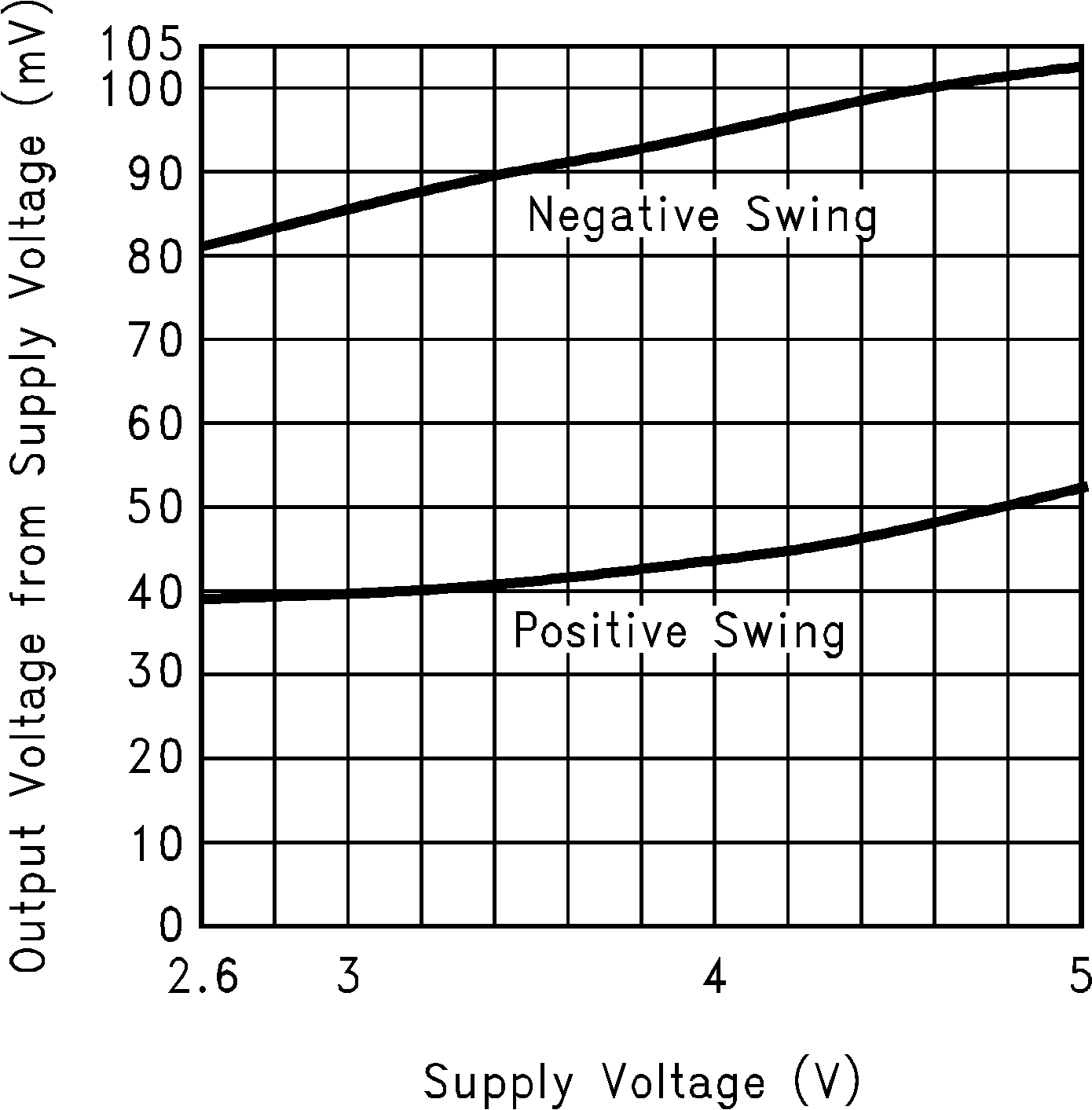Figure 8. Output Voltage Swing vs. Supply Voltage
(RL = 2kΩ)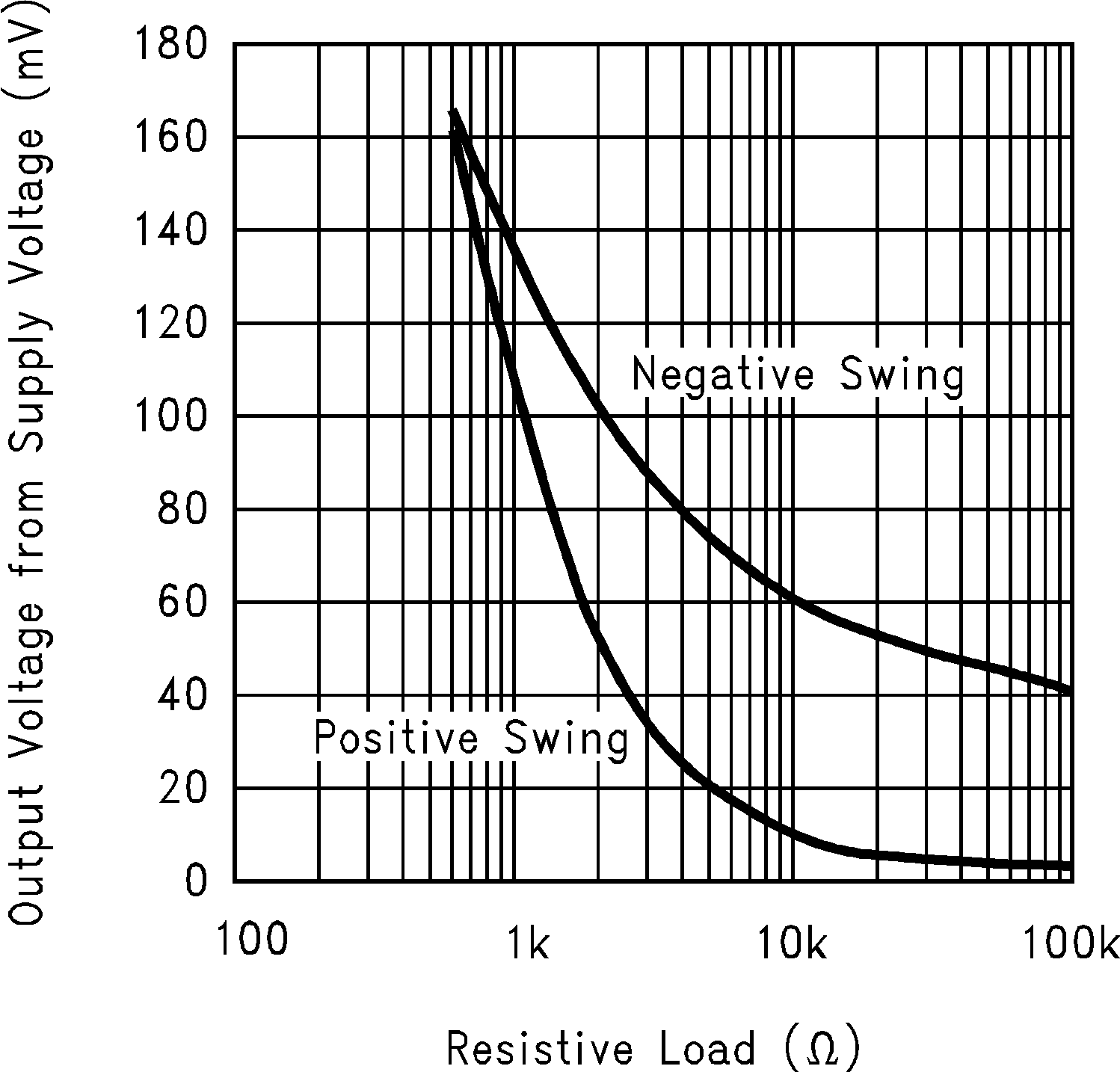Figure 10. Output Voltage Swing vs. Load Resistance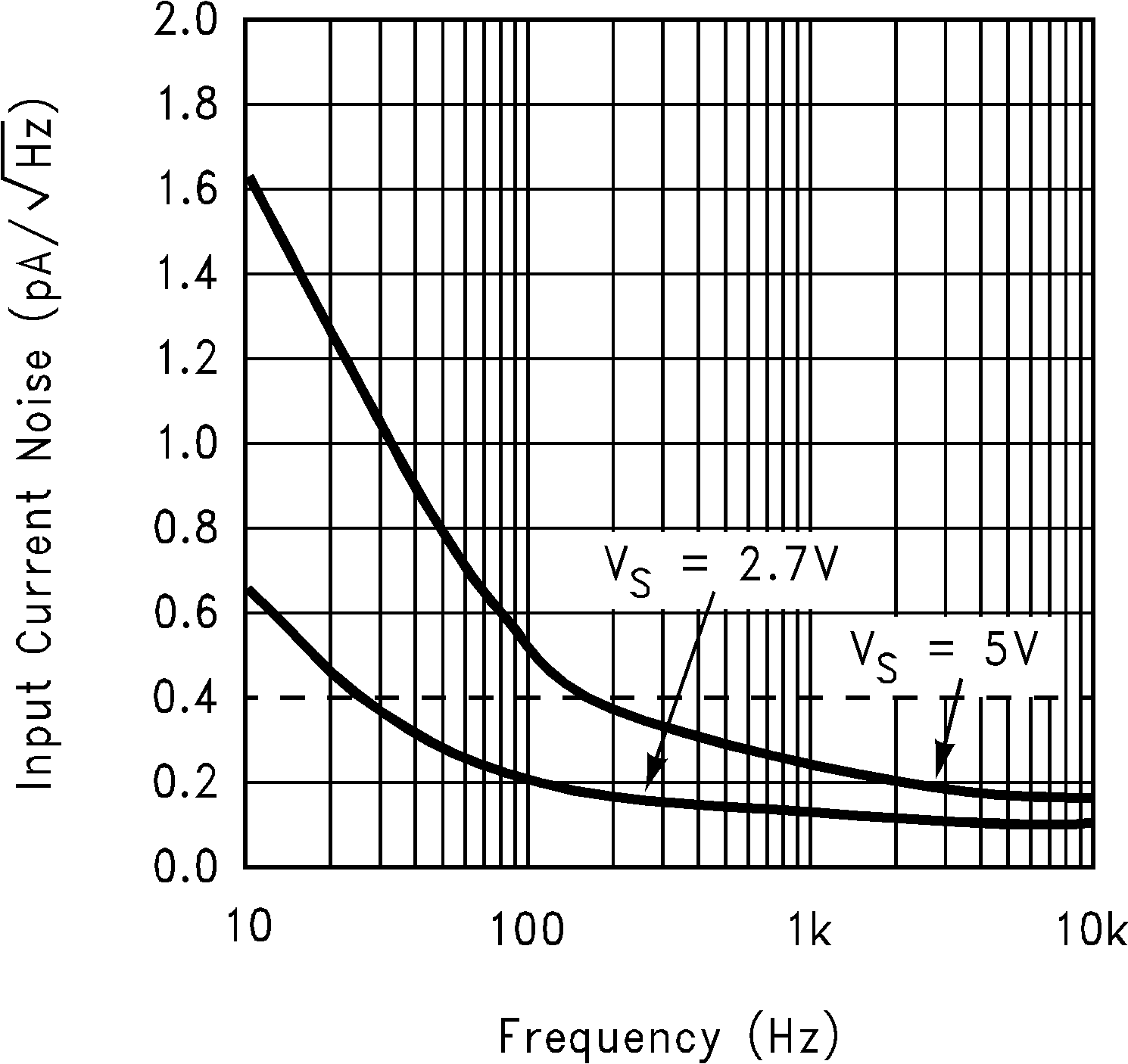Figure 12. Input Current Noise vs. Frequency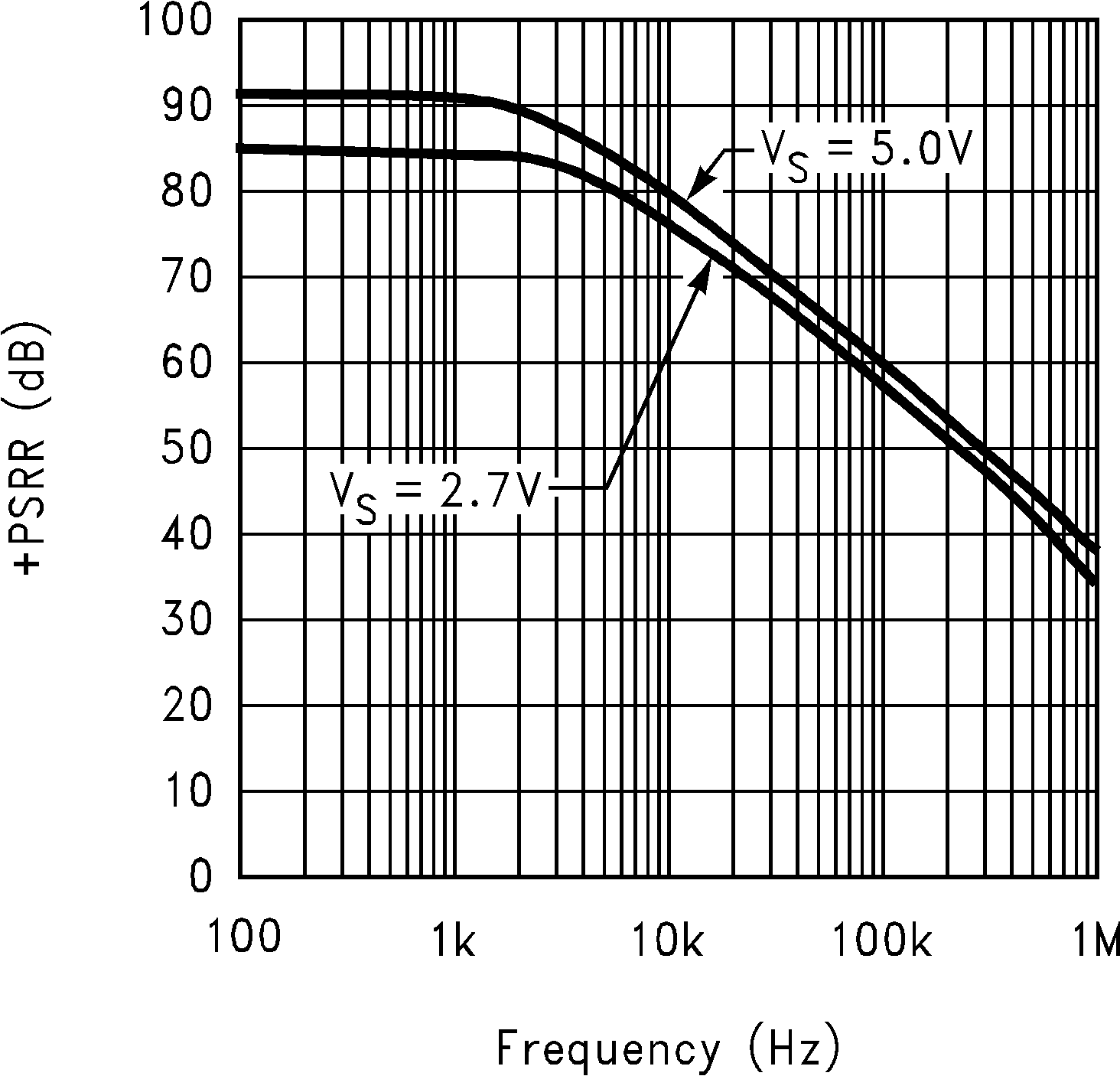Figure 14. +PSRR vs. Frequency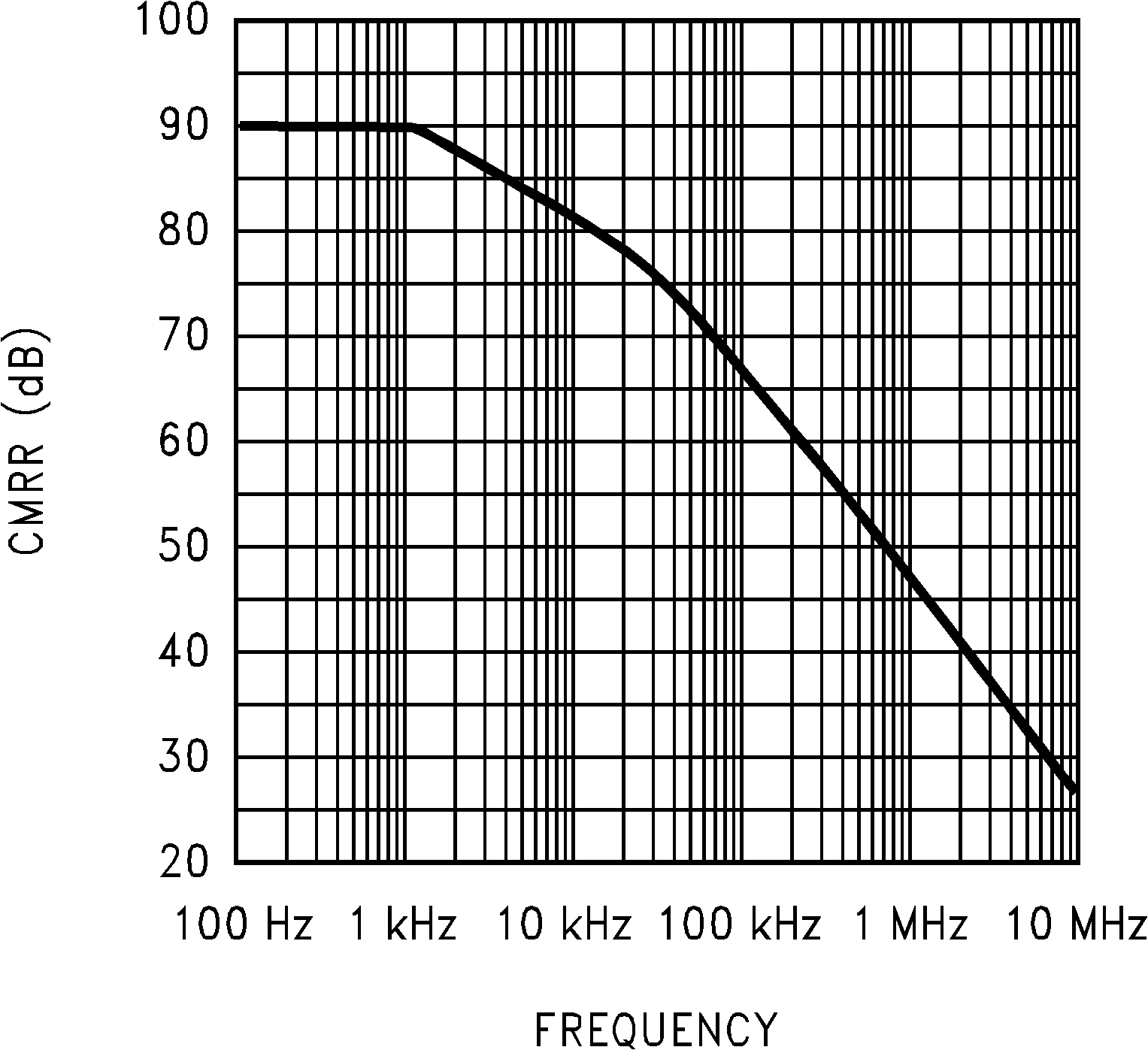Figure 16. CMRR vs. Frequency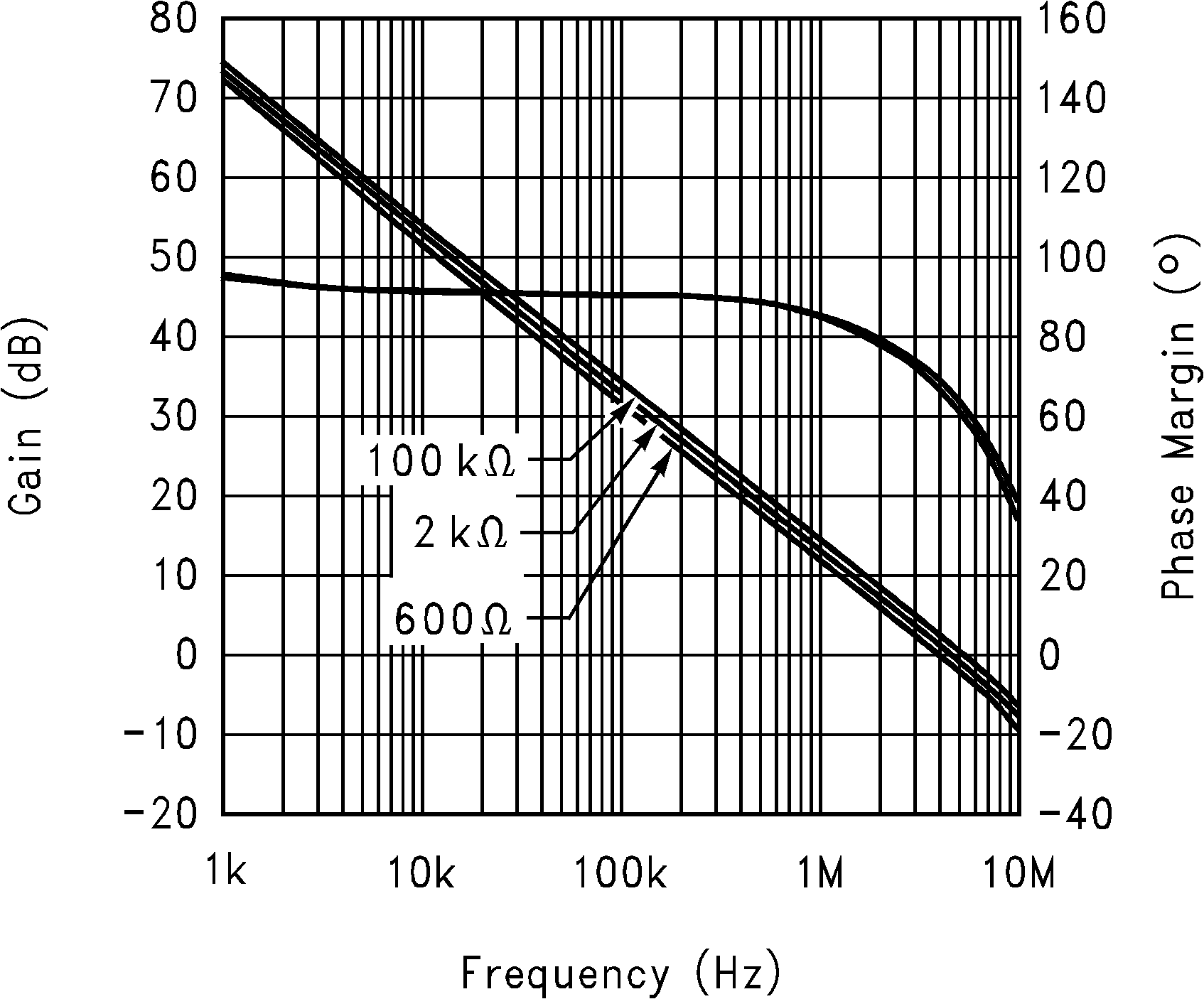Figure 18. Gain and Phase Margin vs. Frequency
(RL = 100kΩ, 2kΩ, 600Ω) at 2.7V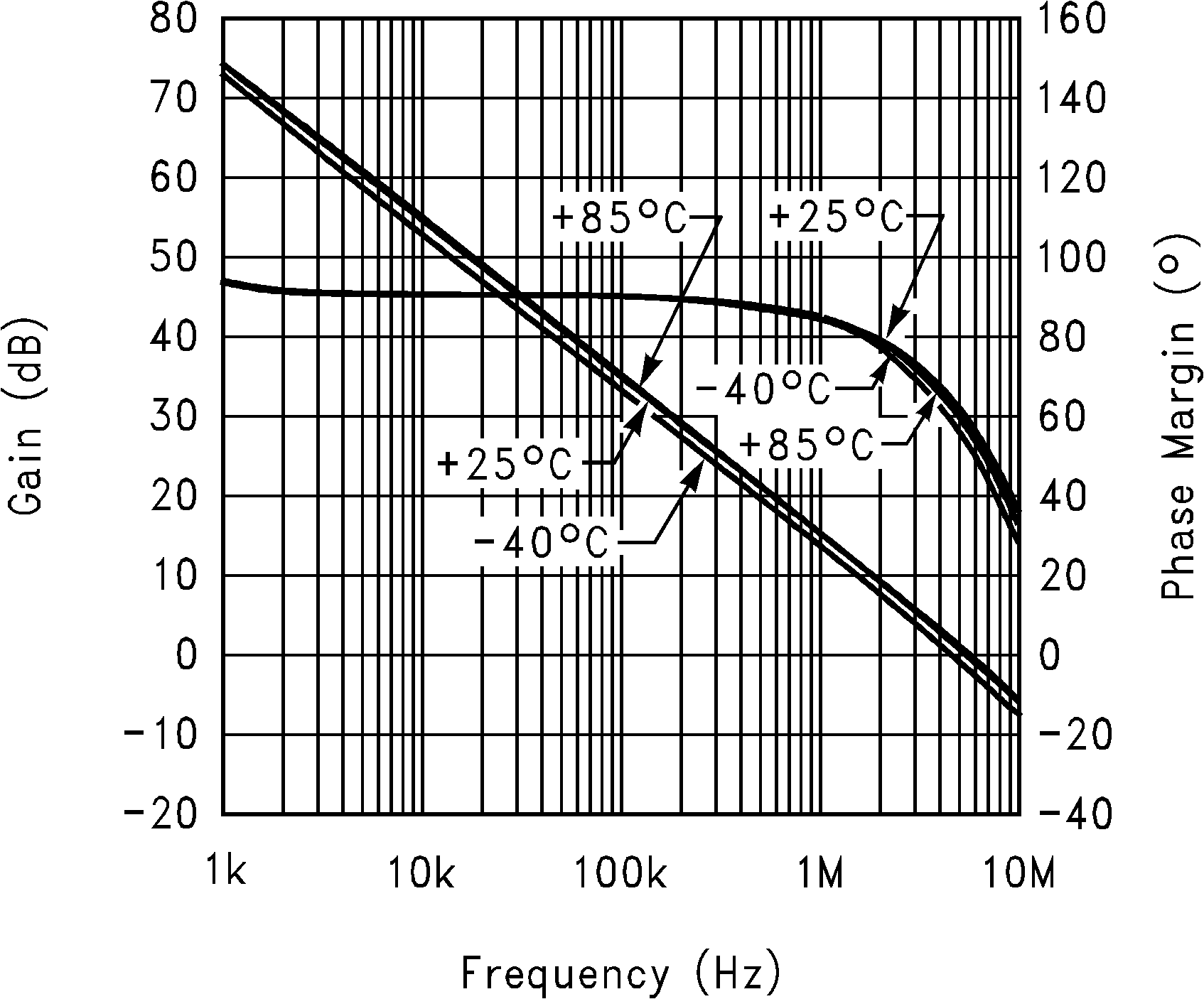Figure 20. Gain and Phase Margin vs. Frequency
(Temp.= 25, -40, 85°C, RL = 10kΩ) at 2.7V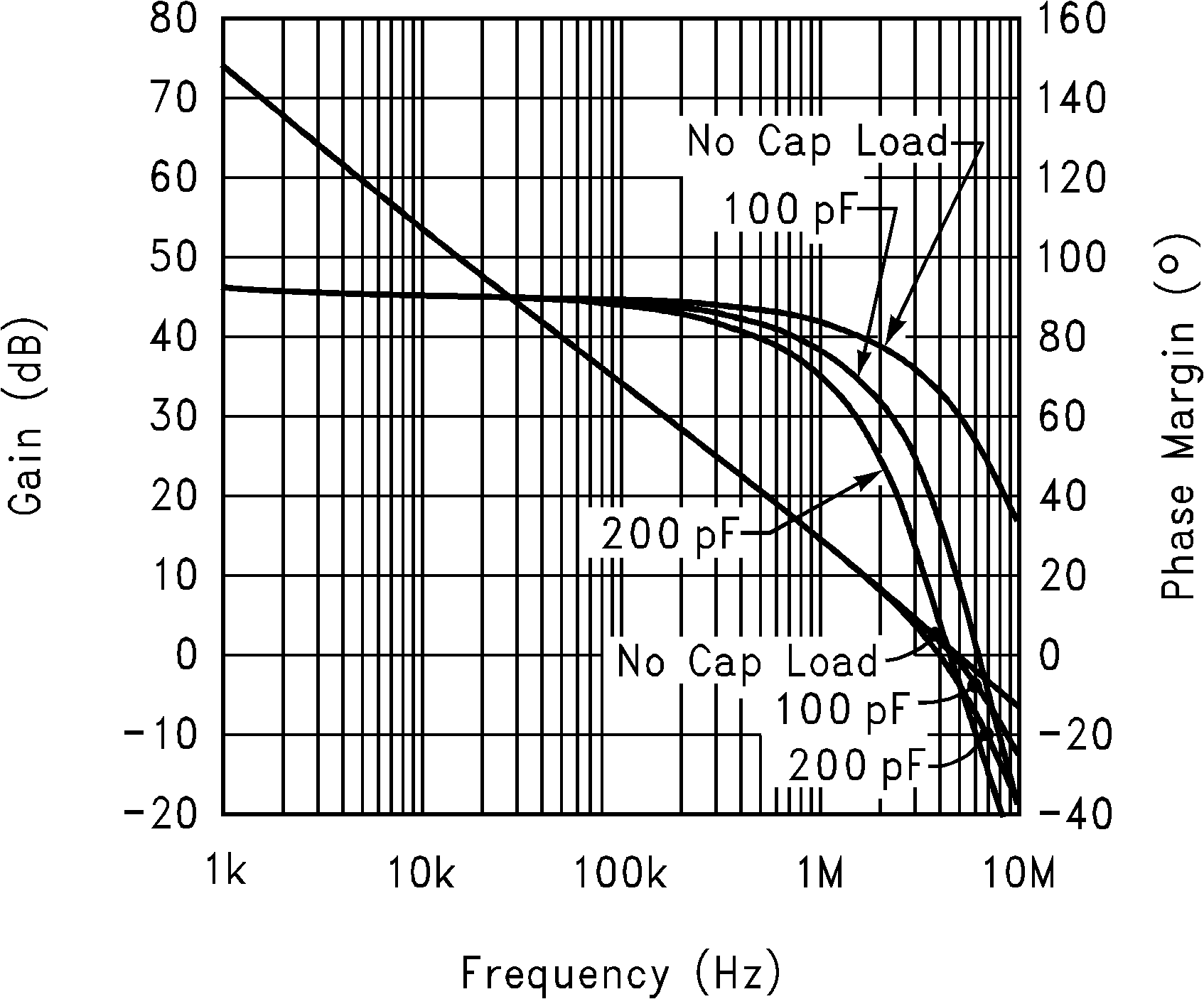Figure 22. Gain and Phase Margin vs. Frequency
(CL = 100pF, 200pF, 0pF, RL = 10kΩ) at 2.7V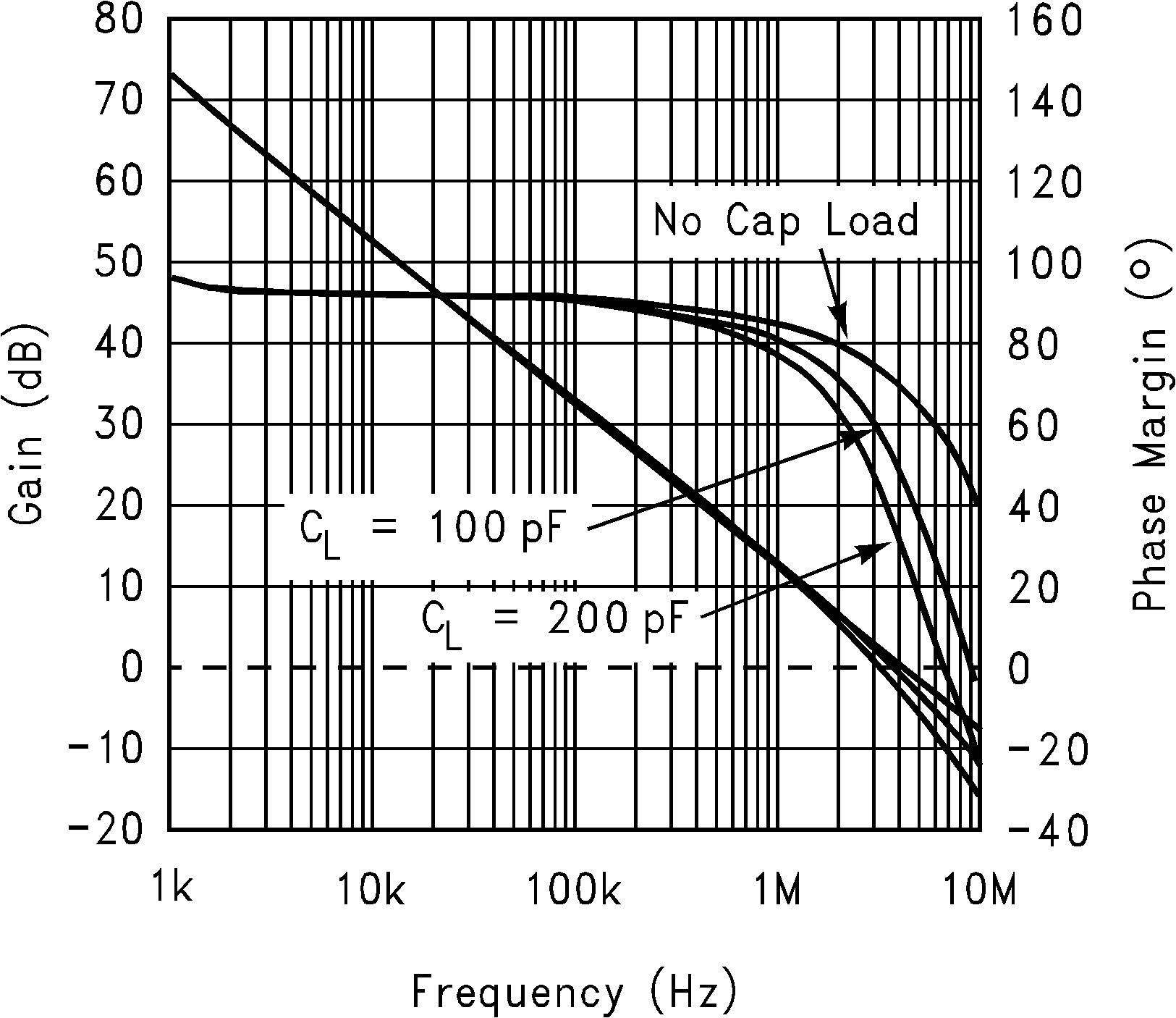Figure 24. Gain and Phase Margin vs. Frequency
(CL = 100pF, 200pF, 0pF RL = 600Ω) at 2.7V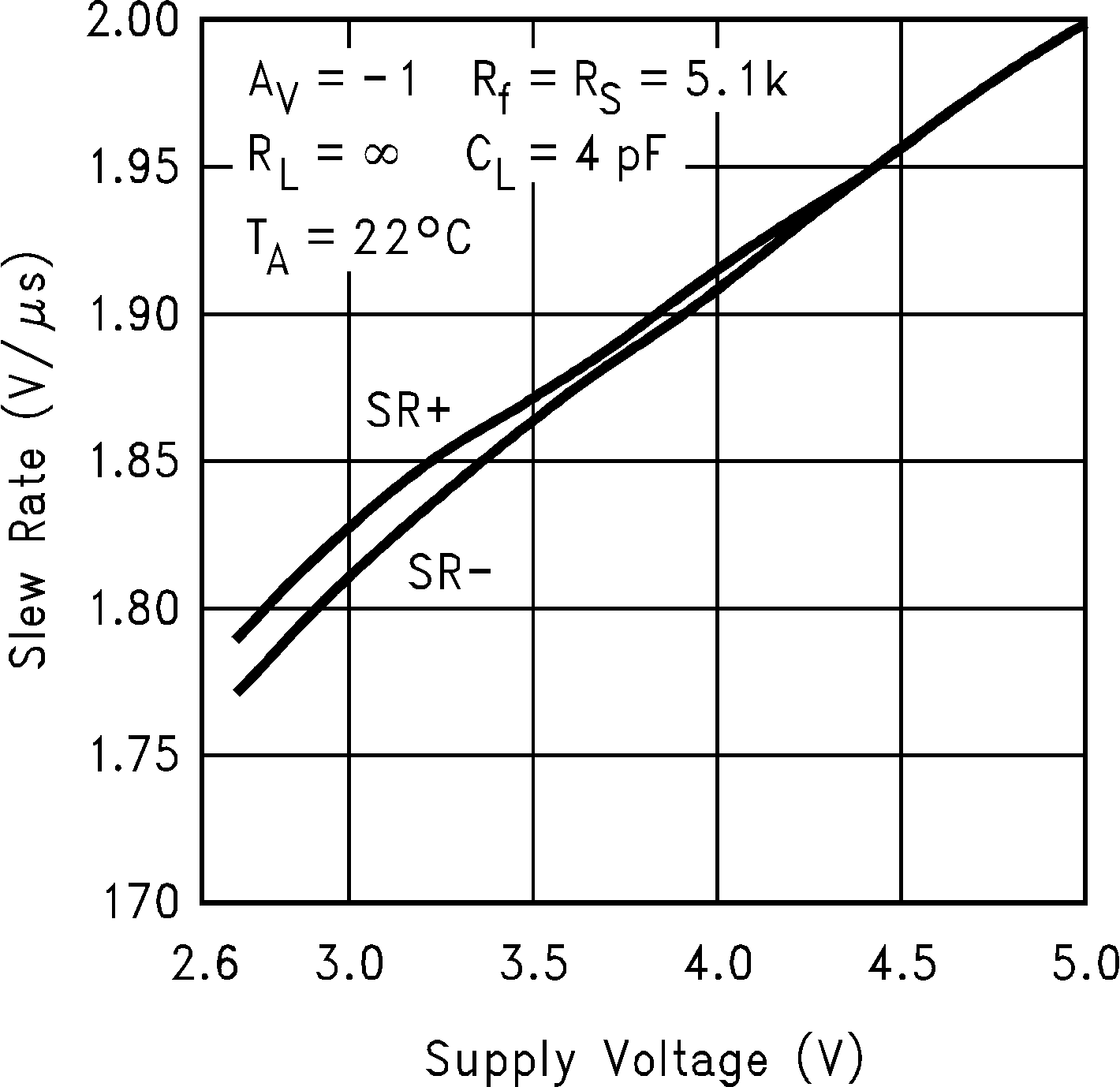Figure 26. Slew Rate vs. Supply Voltage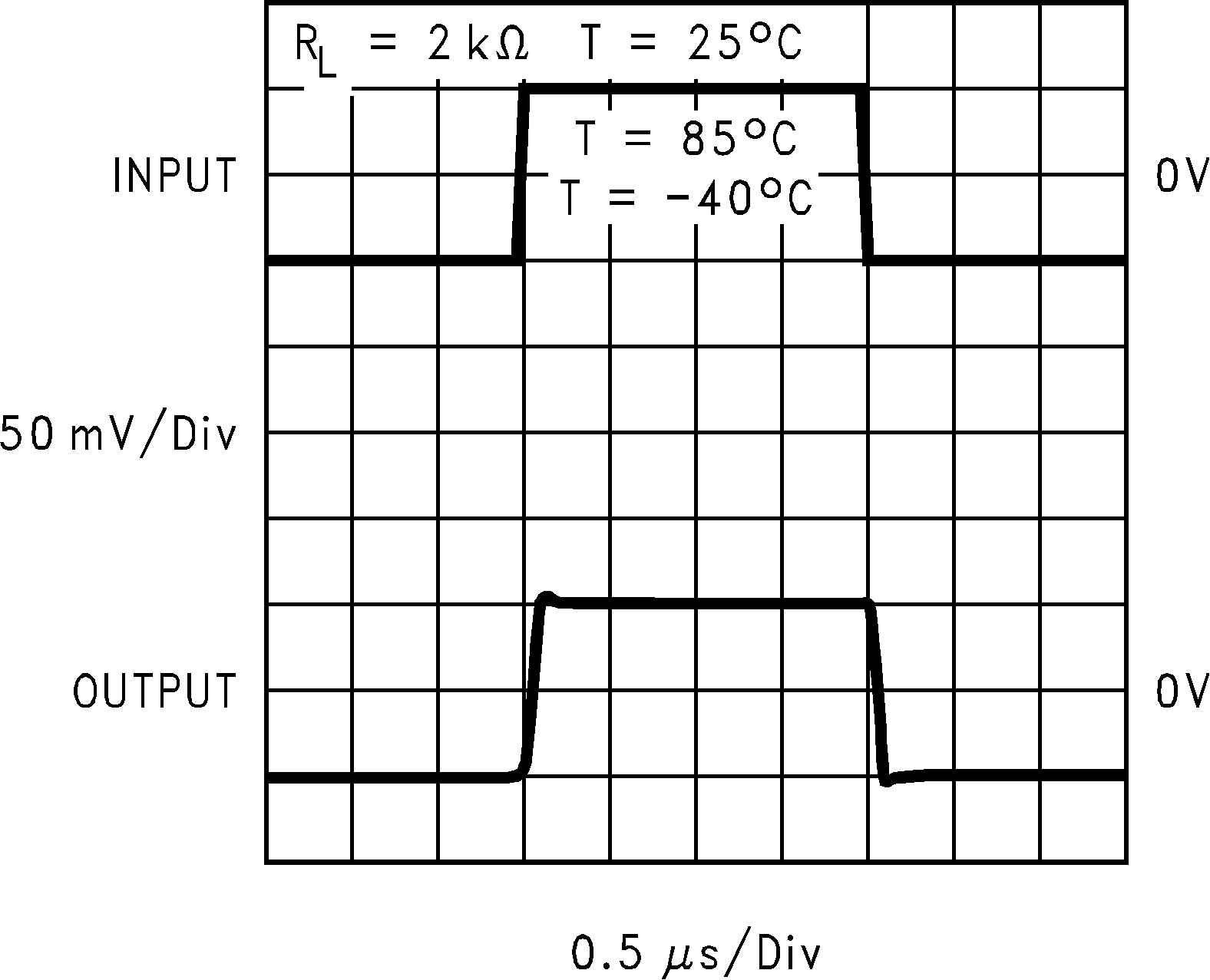Figure 28. Non-Inverting Small Signal Pulse Response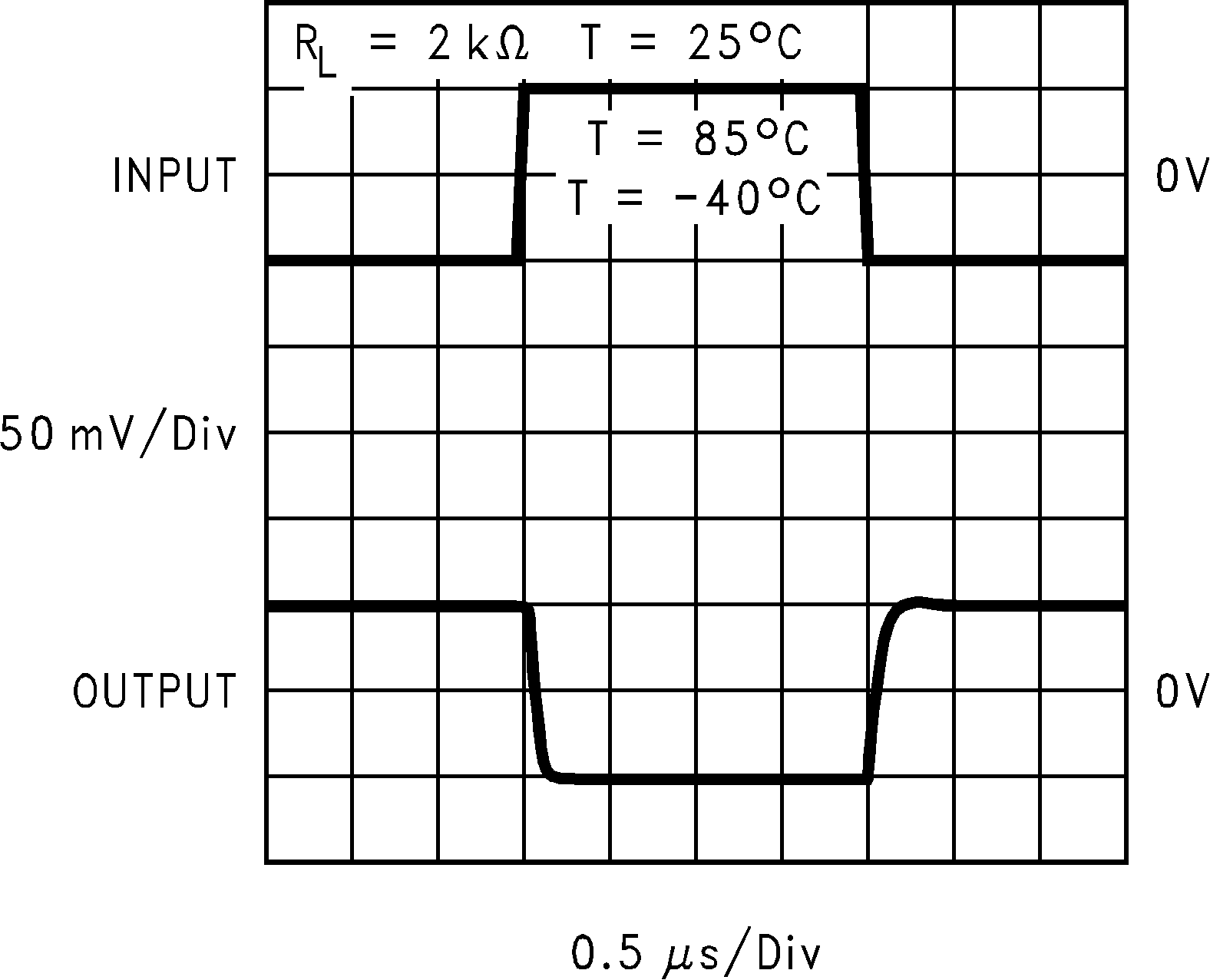Figure 30. Inverting Small Signal Pulse Response